# Equilibrium Shapes with Stress Localisation for Inextensible Elastic Möbius and Other Strips

## Abstract

We formulate the problem of finding equilibrium shapes of a thin inextensible elastic strip, developing further our previous work on the Möbius strip. By using the isometric nature of the deformation we reduce the variational problem to a second-order one-dimensional problem posed on the centreline of the strip. We derive Euler–Lagrange equations for this problem in Euler–Poincaré form and formulate boundary-value problems for closed symmetric one- and two-sided strips. Numerical solutions for the Möbius strip show a singular point of stress localisation on the edge of the strip, a generic response of inextensible elastic sheets under torsional strain. By cutting and pasting operations on the Möbius strip solution, followed by parameter continuation, we construct equilibrium solutions for strips with different linking numbers and with multiple points of stress localisation. Solutions reveal how strips fold into planar or self-contacting shapes as the length-to-width ratio of the strip is decreased. Our results may be relevant for curvature effects on physical properties of extremely thin two-dimensional structures as for instance produced in nanostructured origami.

## Introduction

We do not know who was the first to take a thin flexible strip of paper (papyrus, parchment, birch bark, animal skin, palm leaf or whatever material), to join its ends in space so that the long edges of the strip make a single closed curve and to admire the resulting shape. Certainly it happened a long time ago. However, the mathematical description of such an object was given only in 1858, independently by August Ferdinand Möbius and Johann Benedict Listing. The Möbius strip is not only interesting from an aesthetic or mathematical point of view but also as a generic example of a strip whose equilibrium shape demonstrates principal characteristic features of other elastic ribbons.

Since Möbius the eponymous strip has had a significant impact on human culture. Its intriguing beauty has inspired many artists including M.C. Escher . In engineering, pulley belts are often used in the form of Möbius strips in order to wear ‘both’ sides equally. At a much smaller scale, Möbius strips have recently been formed in ribbon-shaped NbSe3 crystals under certain growth conditions involving a large temperature gradient [55, 56]. The mechanism proposed by Tanda et al. to explain this behaviour is a combination of Se surface tension, which makes the crystal bend, and twisting as a result of bend-twist coupling due to the crystal nature of the ribbon. Gravesen & Willatzen  computed quantum eigenstates of a particle confined to the surface of a developable Möbius strip and compared their results with earlier calculations by Yakubo et al. . They found curvature effects in the form of a splitting of the otherwise doubly degenerate ground state wave function (see also ). Thus qualitative changes in the physical properties of Möbius strip structures (for instance nanostrips) may be anticipated and it is of physical interest to know the exact shape of a free-standing strip. It has also been theoretically predicted that a novel state appears in a superconducting Möbius strip placed in a magnetic field . Möbius strip geometries have furthermore been proposed to create optical fibres with tunable polarisation  and the Möbius strip topology has been suggested for compact resonators that have a resonance frequency that is half that of a cylindrical loop of the same size .

There exist infinitely many realisations of the Möbius strip and attempts have been made to define a unique, ‘canonical’, shape that is in some sense the simplest [42, 44, 47, 48, 61]. One way to approach this problem is to ask for the shape adopted by a free-standing Möbius strip made of a thin material (like paper); see Fig. 1.Footnote 1 In the idealised case when we can neglect the material’s stretching, the shape may be found as that minimising the elastic energy associated with bending. A strip that deforms without stretching has constant Gaussian curvature under deformation. If such a strip is intrinsically flat (i.e., has zero Gaussian curvature) then it deforms into a developable surface: it can be unrolled onto a plane [13, 49]. Sadowsky was probably the first who formulated the problem in this way [44, 45]: find the shape of a Möbius strip by minimisation of the integral of the squared mean curvature over a deformed rectangular domain under the constraint of developability. Sadowsky went further and also derived the equations that describe the equilibrium shape of a thin inextensible elastic strip in the limit of vanishing width . For a strip of finite width, Wunderlich showed how to reduce the variational problem to a one-dimensional one: he carried out analytical integration over the width of the strip so that the variational problem reduces to minimisation of the remaining one-dimensional integral over the centreline of the strip . He didn’t derive the Euler–Lagrange equilibrium equations for this reduced variational problem. It was proven recently that the Sadowsky functional is the Γ-limit of the Wunderlich functional for centrelines with non-vanishing curvature .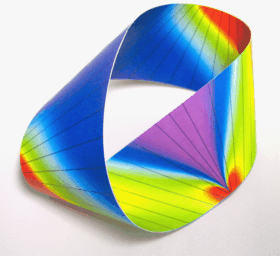Fig. 1

An alternative approach has been to model a narrow elastic Möbius strip as a thin inextensible elastic rod with non-circular cross-section taken in the limit where one of the bending stiffnesses tends to infinity . Although the centreline shapes obtained using this approach are superficially in good agreement with those of a real narrow Möbius strip, such a model fails to reproduce the generic characteristic features of the surface of twisted inextensible strips.

Approximate equations for deformations of wide strips were derived in the mid-1950s by Mansfield [34, 35]. These equations predict the distribution of generators of the developable surface while ignoring the actual three-dimensional geometry. This work was followed up in , where localisation of stresses at two diagonally opposite corners was found for a strip in its first buckling mode. The actual shape of the strip was not computed.

The Euler–Lagrange equations for the Sadowsky functional describing equilibria of a narrow developable strip were derived in . Some explicit solutions of the same variational problem were presented in [8, 22]. Folded narrow annular strips were studied in .

The geometrically exact equilibrium equations for an elastic developable strip were presented in  together with their numerical solution for Möbius strips of various aspect ratios. Here we give a more detailed discussion of the theory of developable strips including a more self-contained analysis of the variational problem and a complete formulation, and solution, of the boundary-value problem for the Möbius strip. We then extend the work to closed one- and two-sided strips of different topology.

## Geometry of a Developable Strip

Consider an inextensible ribbon that, when developed onto a plane, makes a strip that is bounded by two parallel straight lines. By the developability property, the strip, however deformed, can be reconstructed from its centreline, i.e., the line that is equidistant from both parallel lines in the intrinsic geometry of the strip. We denote this line by $$\boldsymbol{r}(s) \in \mathbb{R}^{3}$$, where s∈[0,L] is arclength along the curve and L is its length. We assume that r(s) is a regular curve of differentiability class C 3.

Let t=r′ be the unit tangent vector (here and in what follows a prime denotes the derivative with respect to s). In points where the curvature κ=|t′|≠0 we define the unit principal normal n=t′/κ and the unit binormal b=t×n. Like any regular curve in $$\mathbb{R}^{3}$$, r(s) is determined, up to Euclidean motion, by its curvature κ(s) and torsion τ(s)=−b′(s)⋅n(s).

A non-planar developable surface is either (part of) a cylinder, a cone or a so-called rectifying developable . An analytical flat Möbius strip can only be of the latter type  (analyticity rules out Sadowsky’s  construction of a Möbius strip from planar and cylindrical pieces). Given a curve with non-vanishing curvature there is a unique rectifying developable on which this curve is a geodesic curve. The unit normal N to the surface at the curve is then aligned with the principal normal to the curve and the surface is the envelope of rectifying planes orthogonal to the principal normal to the curve. Then

\begin{aligned} \begin{aligned} &\boldsymbol{x}(s,t) = \boldsymbol{r}(s) + t \bigl[\boldsymbol{b}(s)+\eta(s)\boldsymbol{t}(s) \bigr],\\ &\tau(s)=\eta(s)\kappa(s), \quad s=[0,L],\ t=[-w,w], \end{aligned} \end{aligned}
(1)

is a parametrisation of a rectifying developable strip with centreline r and width 2w . The straight lines s=const. are the generators of the surface. They make an angle β=arctan(1/η) with the positive tangent direction of the curve r(s) (see Fig. 2). The short edges s=0 and s=L of the strip in particular are generators and for closure of the strip we require |η(0)|=|η(L)| (η(L)=η(0) [parallelogram] for a two-sided surface and η(L)=−η(0) [isosceles trapezium] for a one-sided surface).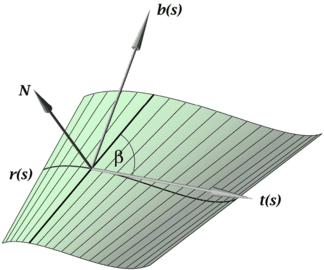Fig. 2

We compute the first fundamental form of the surface coordinate patch given by Eq. (1):

$$\mathbb{I}=\mathrm{d} \boldsymbol{x} \cdot \mathrm{d} \boldsymbol{x} = E\,\mathrm{d} s^2 + 2F\,\mathrm{d} s \,\mathrm{d} t + G\,\mathrm{d}t^2,$$

where

\begin{aligned} E =& \boldsymbol{x}_s \cdot \boldsymbol{x}_s = \bigl(1+t \eta'\bigr)^2, \\ F =& \boldsymbol{x}_s \cdot \boldsymbol{x}_t = \eta\bigl(1+t \eta'\bigr), \\ G =& \boldsymbol{x}_t \cdot \boldsymbol{x}_t = 1+\eta^2 . \end{aligned}

The area element is $$\mathrm{d}\sigma =\|\boldsymbol{x}_{s} \times \boldsymbol{x}_{t}\|\,\mathrm{d}t\, \mathrm{d}s=\sqrt{EG-F^{2}}\,\mathrm{d}t \,\mathrm{d}s = (1+t\eta')\,\mathrm{d}t\,\mathrm{d}s$$. The unit normal to the surface $$\boldsymbol{N} (s,t) = \frac{\boldsymbol{x}_{s} \times \boldsymbol{x}_{t}}{\|\boldsymbol{x}_{s} \times \boldsymbol{x}_{t}\|} = -\boldsymbol{n}(s)$$ is constant along any generator. We also need the second fundamental form defined by

$$\mathbb{II}=-\mathrm{d} \boldsymbol{x} \cdot \mathrm{d} \boldsymbol{N} = e\,\mathrm{d} s^2+ 2f\,\mathrm{d} s \,\mathrm{d} t + g\,\mathrm{d}t^2,$$

where

\begin{aligned} e =& -\boldsymbol{N}_s \cdot \boldsymbol{x}_s = -\kappa \bigl(1+t \eta'\bigr) , \\ f =& -\boldsymbol{N}_s \cdot \boldsymbol{x}_t = 0, \\ g =& -\boldsymbol{N}_t \cdot \boldsymbol{x}_t = 0 . \end{aligned}

We can now compute the shape operator (or Weingarten map) S, i.e., the linear operator on the tangent plane defined by S(T)=− T N, where T is a unit tangent vector to the surface . S(T) is the gradient of the unit normal to the surface in the tangent direction T. It therefore encodes information about the curvature of the surface. In fact, the eigenvalues of the shape operator at each point are the principal curvatures at this point and the eigenvectors are the principal directions. We have S(x s )=−N s , S(x t )=−N t , and hence in the basis (x s ,x t ),

$$S=\left(\begin{array}{c@{\quad}c} E & F \\ F & G \end{array}\right)^{-1} \left(\begin{array}{c@{\quad}c} e & f \\ f & g \end{array}\right) = \frac{1}{EG-F^2} \left(\begin{array}{c@{\quad}c} eG-fF & fG-gF \\ fE-eF & gE-fF \end{array}\right)= \left(\begin{array}{c@{\quad}c} \frac{-\kappa (1+\eta^2)}{1+t\eta'} & -\kappa \eta \\ 0 & 0 \end{array}\right).$$

We then compute the Gaussian curvature as

$$K:=\operatorname{det}S=\kappa_1\kappa_2=\frac{eg-f^2}{EG-F^2}=0 ,$$

confirming that it vanishes identically, while for the mean curvature we find

$$H:=\frac{1}{2}\operatorname{tr}S=\frac{1}{2}(\kappa_1+ \kappa_2)= \frac{eG+gE-2fF}{2(EG-F^2)}=-\frac{\kappa(1+\eta^2)}{2(1+t\eta')} ,$$
(2)

where κ 1 and κ 2 are the two principal curvatures of the surface.

As to the space-curve geometry of the centreline r we recall the following. After choosing a coordinate system we may identify the orientations of the Frenet–Serret frame {t,n,b} attached to r with elements of the group of orthogonal 3×3 matrices:

$$R(s) := \bigl(\boldsymbol{t}(s), \boldsymbol{n}(s), \boldsymbol{b}(s)\bigr)\in \mathit{SO}(3) .$$

This defines a skew-symmetric 3×3 matrix in the Lie algebra $$\mathfrak{so}(3)$$ as follows:

$$\mathsf{\widehat{\omega}} = R^\intercal R',$$
(3)

where we have introduced the ‘hat’ isomorphism between skew-symmetric matrices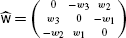in $$\mathfrak{so}(3)$$ and axial (or rotation) vectors w=(w 1,w 2,w 3) in $$\mathbb{R}^{3}$$.Footnote 2 By definition of the Frenet–Serret frame, we have ω 1=τ, ω 2=0, ω 3=κ. For a curve on a surface we define its Darboux frame {t,N,t×N}. The rotation (Darboux) vector has components (τ g ,κ g ,κ N ), which have their own names, geodesic torsion, geodesic curvature and normal curvature, respectively. They relate to the curvature and torsion of the curve as τ g =τ+χ′, κ g =κsinχ, κ N =κcosχ, where χ measures the angle from n to N . Here we consider only the case of a geodesic centreline and we thus have n=−N, hence χ=π and τ g =τ=ω 1, κ g =0=ω 2, κ N =−κ=−ω 3.

The Frenet–Serret frame is discontinuous at an inflection point where the normal n (and hence the binormal b) flips (n→−n). For a continuous description through an inflection point we can however use a generalised Frenet–Serret frame (whose normal and binormal are plus or minus the normal and binormal of the Frenet–Serret frame and such that the frame is continuous through an inflection point) and let κ be the signed curvature [39, 42].

### Edge of Regression

The asymptotic completion of a developable strip is defined as the surface obtained by extending all generators to infinity in both directions, i.e., taking t in all of $$\mathbb{R}$$ in Eq. (1). From Eq. (2) we see that the mean curvature H becomes singular if the parameter t in Eq. (1) equals −1/η′. If η′=0 then there is no singularity on the extended generator in the asymptotic completion. We call points on the centreline where η′=0, ‘cylindrical’. At such points the mean curvature H is independent of t, so the principal curvatures are constant along the local generator. Away from cylindrical points we can define the curve $$\boldsymbol{x}_{e}(s) = \boldsymbol{r}(s) -\frac{1}{\eta'(s)} [\boldsymbol{b}(s)+\eta(s)\boldsymbol{t}(s) ]$$, which is called the edge of regression. When η′ changes sign the edge of regression jumps within the asymptotic completion from one side of the centreline to the other. (For a graphical representation the reader may wish to look ahead to Fig. 10 for the case of a Möbius strip.) The strip cannot be wider than the critical value of t, i.e., we require that w|η′|≤1. The developable surface is then the envelope of the tangents to the edge of regression (i.e., of the generators of the surface) and is therefore also the tangent developable. This envelope meets the edge of regression in two sheets that form cusps in the normal plane to x e .

The edge of regression may have its own singularities. By differentiation we find $$\boldsymbol{x}'_{e}(s)=\frac{\eta''(s)}{\eta'^{2}(s)} [\boldsymbol{b}(s)+\eta(s)\boldsymbol{t}(s) ]$$. If η″=0 the tangent to the edge of regression is discontinuous. This corresponds to a cusp point on the curve and a swallow tail singularity of the asymptotic completion of the strip. We call isolated points of the centreline where η″=0, ‘conical’. Clearly, for a smooth centreline, there must be a conical point between any pair of cylindrical points.

## The Energy Functional

The bending energy for an arbitrary Kirchhoff–Love shell of thickness 2d can be written as the following integral over the surface of the strip [12, 38, 63]:

$$U=\frac{D}{2}\iint_\varOmega \bigl[\nu \bigl(\operatorname{tr}(\Delta S) \bigr)^2 + (1-\nu) \operatorname{tr}\bigl((\Delta S)^2\bigr)\bigr] \, \mathrm{d} \sigma ,$$
(4)

where D=2Yd 3/[3(1−ν 2)] is the flexural rigidity, ν is Poisson’s ratio, Y is Young’s modulus and ΔS=SS 0; here and later the subscript 0 refers to the undeformed reference configuration. By expressing both S and S 0 in principal axes of S 0, we may write

$$S= \left ( \begin{array}{c@{\quad}c} \cos\Delta\beta & -\sin\Delta\beta \\ \sin\Delta\beta & \cos\Delta\beta \end{array} \right ) \left(\begin{array}{c@{\quad}c} \kappa_1 & 0 \\ 0 & \kappa_2 \end{array}\right) \left ( \begin{array}{c@{\quad}c} \cos\Delta\beta & \sin\Delta\beta \\ -\sin\Delta\beta & \cos\Delta\beta \end{array} \right ),\quad S_0= \left(\begin{array}{c@{\quad}c} \kappa_{1,0} & 0 \\ 0 & \kappa_{2,0} \end{array}\right),$$

where Δβ=ββ 0 is the angle between the principal curvature axes in the deformed and undeformed states. Equation (4) then becomes 

$$U=D\iint_\varOmega \bigl[(1+\nu) (\Delta H)^2 + (1-\nu) \bigl(( \Delta A)^2 + 4 A A_0 \sin^2 \Delta\beta \bigr)\bigr] \,\mathrm{d}\sigma ,$$
(5)

where ΔH=HH 0 and $$A=\sqrt{H^{2}-K}$$.

In our case of a developable surface obtained by isometric deformation from its unstressed state we have zero Gaussian curvature K (the undeformed surface must be flat, although not necessarily planar). This means that one of the principal curvatures is zero (say κ 2=0) and we may write

$$U=\frac{D}{2}\iint_\varOmega \bigl[(\kappa_1- \kappa_{1,0})^2+2(1-\nu)\kappa_1 \kappa_{1,0} \sin^2 \Delta\beta\bigr] \, \mathrm{d}\sigma .$$
(6)

The fact that the strip is fully determined by its centreline suggests reduction of this double integral to a single integral over the centreline. However, this reduction looks intractable for an arbitrary undeformed shape given by κ 1,0. We develop the general theory a little further, and consider two special cases, in Appendix A, but here we proceed by assuming that the strip is planar when relaxed. Thus we set κ 1,0=0 and have, with κ 1=2H,

$$U=\frac{D}{2}\int_{0}^{L} \int _{-w}^{w} \frac{\kappa^2 (1+\eta^2)^2}{1+t\eta'} \,\mathrm{d}t \,\mathrm{d}s .$$
(7)

The t-integration can be carried out analytically , and we arrive at

$$U=Dw\int_0^L h\bigl(\kappa,\eta, \eta'\bigr) \, \mathrm{d}s$$
(8)

with

\begin{aligned} \begin{aligned} &h\bigl(\kappa,\eta,\eta'\bigr)=\kappa^2 \bigl(1+ \eta^2 \bigr)^2 V\bigl(w\eta'\bigr),\\ &V\bigl(w\eta'\bigr)=\frac{1}{2w\eta'}\log \biggl( \frac{1+w\eta'}{1-w\eta'} \biggr) . \end{aligned} \end{aligned}
(9)

Note that for strips with no intrinsic curvature, equilibrium shapes do not depend on the material properties: Young’s modulus is a simple factor and Poisson’s ratio does not enter the energy expression. Also note that in the limit of narrow strips, ′→0, we have V(′)→1 and no derivative enters the integrand in Eq. (8) (cf. [44, 45]). The integrand h reduces to κ 2 of the planar Euler elastica if η≡0, the surface then being cylindrical with the generator everywhere perpendicular to the centreline r.

For solutions of closed strips or strips with fixed end points we impose a constant end-to-end distance constraint by adding the following integral expression to the bending energy:

$$W=-\boldsymbol{F} \cdot \bigl[\boldsymbol{r}(L) - \boldsymbol{r}(0)\bigr] = -\int_0^L \boldsymbol{F} \cdot \boldsymbol{t} \,\mathrm{d} s,$$

where we have used inextensibility of the centreline, i.e., t=r′, and F is a (constant) Lagrange multiplier (with the physical meaning of an internal force). Finally, we have to impose the constraint that the centreline is geodesic (straight in the intrinsic geometry), i.e., ω 2=κ g ≡0. We enforce this constraint by adding the integral $$T=\int_{0}^{L} M_{2} \omega_{2} \,\mathrm{d} s$$, where M 2=M 2(s) is another (local) Lagrange multiplier.

In conclusion, equilibrium shapes of a thin, inextensible and intrinsically planar strip are given by stationary points of the functional

$$\mathcal{A}=\bar{U}+W+T=\int_0^L\mathcal{L}\, \mathrm{d}s, \quad \mbox{with } \mathcal{L}=h+M_2 \omega_2-\boldsymbol{F} \cdot \boldsymbol{t},$$
(10)

where $$\mathcal{L}$$ is the Lagrangian of the problem and $$\bar{U}=U/(Dw)$$ (all force and moments in the rest of the paper are thus normalised by Dw). This represents a 1D variational problem on a curve in $$\mathbb{R}^{3}$$ cast in Euclidean invariant form.

Although we are here interested in closed-strip solutions, the functional $$\mathcal{A}$$ can also be used to study open-strip solutions. If for such open strips the ends are free to move relative to each other then W represents the work done by any applied end force F. However, since we use the parametrisation of Eq. (1) in the t-integration to obtain the one-dimensional integral (8), the short edges of the strip must be generators and therefore straight. This is quite natural for fixed ends (held by a straight clamp covering the entire edge of the strip) but constitutes a restriction on deformations considered for strips with free ends.

## Variational Principle and Equilibrium Equations

### Variational Principle

To derive the equilibrium equations for the functional $$\mathcal{A}$$ we follow the recent higher-order variational approach of . This approach yields equations in a particularly elegant and transparent geometrical form if we view the Lagrangian $$\mathcal{L}$$ as a function of the rotation matrix R and its derivatives, i.e., as defined on the (higher-order) tangent bundle of the symmetry group of our problem, the Lie group SO(3). The theory in  then gives a symmetry-reduced variational problem with Euler–Lagrange equations in Euler–Poincaré form. Similar treatments of higher-order variational problems on curves can be found in [24, 52].

We can transform $$\mathcal{L}$$ into suitable form by expressing κ, η, η′ and ω 2 in terms of the Frenet–Serret frame viewed as a rotation matrix, R (see Sect. 2). The result is the second-order Lagrangian $$\mathcal{L}= \mathcal{L}_{\boldsymbol{F}}(R,R',R''):T^{(2)}\mathit{SO}(3) \to \mathbb{R}$$, where T (2) SO(3) is the second-order tangent bundle and $$\boldsymbol{F} \in \mathbb{R}^{3}$$ is regarded as a parameter. The functional $$\mathcal{A}$$ may then be written as

$$\mathcal{A} = \int_0^L \mathcal{L}_{\boldsymbol{F}} \bigl(R, R', R''\bigr) \, \mathrm{d} s = \int_0^L \bar{\mathcal{L}}\bigl(R, R', R''\bigr) \, \mathrm{d} s -\int _0^L \boldsymbol{F} \cdot \boldsymbol{t} \, \mathrm{d} s,$$
(11)

where t=R e 1 and $$\bar{\mathcal{L}}$$ collects all terms not depending on F. The parameter-dependent Lagrangian $$\mathcal{L}_{\boldsymbol{F}}$$ is not invariant under SO(3) because of the W term, which is only invariant under rotations S 1 about F. Thus F breaks SO(3) symmetry of $$\mathcal{L}_{\boldsymbol{F}}$$. However, if we view $$\mathcal{L}=\mathcal{L}_{\boldsymbol{F}}$$ as a function defined on $$T^{(2)}\mathit{SO}(3) \times \mathbb{R}^{3}$$ with SO(3) acting by left multiplication on both T (2) SO(3) and the parameter manifold $$\mathbb{R}^{3}$$ then this $$\mathcal{L}$$ is left-invariant under SO(3). We are then in the situation of Sect. 3.3 of  and can apply Euler–Poincaré reduction for Lie groups acting on manifolds to obtain a reduced variational principle.

We consider curves in SO(3) with given end point conditions R(0), R′(0), R(L), R′(L) (these fix the end generators in space). According to Hamilton’s principle such a curve is an extremal of $$\mathcal{A}$$ ($$\delta\mathcal{A}=0$$) under variations of the curve that vanish at the end points, i.e., δR(0)=δR′(0)=δR(L)=δR′(L)=0, while F is held fixed, if and only if it is a solution of the Euler–Lagrange equations. Here we have used the usual notation for infinitesimal variations of a field variable v(s): for a smooth one-parameter family of curves v ϵ (s) we write $$\delta v(s)=\frac{\mathrm{d}}{\mathrm{d} \epsilon}v_{\epsilon}(s)|_{\epsilon=0}$$.

The reduced variational principle is

$$\delta\int_0^L l\bigl( \mathsf{\omega}, \mathsf{\omega}', \mathsf{F}\bigr) \, \mathrm{d} s =\delta \biggl( \int_0^L {\bar{l}}\bigl( \mathsf{\omega}, \mathsf{\omega}'\bigr) \, \mathrm{d} s - \int_0^L \mathsf{F}\cdot\mathsf{t} \, \mathrm{d} s \biggr)=0$$
(12)

for $$l:~2\mathfrak{so}(3) \times \mathbb{R}^{3} \to \mathbb{R}$$ given by $${l}( \mathsf{\omega}, \mathsf{\omega}', \mathsf{F})= \mathcal{L}_{\boldsymbol{F}}(R, R', R'')$$, i.e., l is just $$\mathcal{L}_{\boldsymbol{F}}$$ expressed in different variables, and the constrained variations $$\delta\mathsf{\omega}$$ of $$\mathsf{\omega}$$ and $$\delta\mathsf{\omega}'=(\delta\mathsf{\omega})'$$ of $$\mathsf{\omega}'$$ of the form $$\delta\mathsf{\omega} = \mathsf{\varpi}' + \mathsf{\omega} \times \mathsf{\varpi}$$ with $$\mathsf{\varpi}$$ another axial vector and $$\mathsf{\omega} \times \mathsf{\varpi} := [ \widehat{\mathsf{\omega}}, \widehat{\mathsf{\varpi}} ]$$, the Lie bracket of $$\mathfrak{so}(3)$$, $$\widehat{\mathsf{\varpi}} = R^{\intercal}(\delta R) \in \mathfrak{so}(3)$$ and $$\delta \mathsf{F} = -\widehat{\mathsf{\varpi}}\mathsf{F}$$. The vanishing variation conditions for the non-reduced functional Eq. (11) imply $$\mathsf{\varpi}(0) = \mathsf{\varpi}'(0)=\mathsf{\varpi}(L) = \mathsf{\varpi}'(L)=\mathsf{0}$$ and therefore $$\delta\mathsf{\omega}(0)=\delta\mathsf{\omega}(L)=\mathsf{0}$$. The reduced variables are $$(\mathsf{\widehat{\omega}},\mathsf{F})= (R^{\intercal}R',R^{\intercal}\boldsymbol{F}) \in \mathfrak{so}(3) \times \mathbb{R}^{3}$$. Note that under this reduction the parameter F acquires field status.

F satisfies the equation

$$(\partial_s + \widehat{\mathsf{\omega}})\mathsf{F}=0,$$
(13)

with initial condition F(0)=R (0)F. The Euler–Lagrange equations for the reduced functional take the Euler–Poincaré form

$$(\partial_s + \widehat{\mathsf{\omega}})\mathsf{M} = \frac{\partial {l}}{\partial \mathsf{F}} \times \mathsf{F} ,$$
(14)

with M defined by

$$\mathsf{M} = \mathcal{E}_{\mathsf{\omega}}(l),$$
(15)

where $$\mathcal{E}_{\zeta}(k) := \frac{\partial {k}}{ \partial \zeta} - \frac{\mathrm{d}}{\mathrm{d}s} (\frac{\partial {k}}{\partial \zeta'} )$$ is the Euler–Lagrange operator for the variable ζ.

For further analysis we rewrite Eqs. (13), (14), (15) as the following system: (a) balance equations for the components of the internal force F=(F 1,F 2,F 3) and moment M=(M 1,M 2,M 3) expressed in the Frenet–Serret frame [24, 52]

\begin{aligned} \mathsf{F}'+\mathsf{\omega}\times\mathsf{F} =&\mathsf{0}, \end{aligned}
(16)
\begin{aligned} \mathsf{M}'+\mathsf{\omega}\times\mathsf{M}+\mathsf{t}\times \mathsf{F} =&\mathsf{0}, \end{aligned}
(17)

and (b) the ‘constitutive’ equations

$$M_j = \frac{\partial {l}}{\partial \omega_j} - \frac{\mathrm{d}}{\mathrm{d}s} \frac{\partial {l}}{\partial \omega'_j}, \quad j=1,2,3.$$
(18)

Here we have used that $$\frac{\partial {l}}{\partial \mathsf{F}} = -\mathsf{t} = -\mathsf{e}_{1}$$, e 1≡(1,0,0).

The equations have |F|2 and FM as first integrals.

### Equations in the Original Variables κ, η

We note that Eq. (18) for j=2 is trivial. For j=1 and 3 we use a contact transformation (ω 1,ω 3)=(τ,κ)→(η,κ)  to obtain

\begin{aligned} M_1 =& \frac{\partial\eta}{\partial\omega_1}\mathcal{E}_\eta(h) = \frac{1}{\kappa} \mathcal{E}_\eta(h) , \end{aligned}
(19)
\begin{aligned} M_3 =& \frac{\partial h}{\partial\omega_3} + \frac{\partial\eta}{\partial\omega_3}\mathcal{E}_\eta(h) = \frac{\partial h}{\partial\kappa} - \frac{\eta}{\kappa} \mathcal{E}_\eta(h), \end{aligned}
(20)

or, in more compact form,

\begin{aligned} \partial_\kappa h-\eta M_1-M_3 =& 0, \end{aligned}
(21)
\begin{aligned} \mathcal{E}_\eta(h)-\kappa M_1 =& 0 \end{aligned}
(22)

(cf.  where the signs of moments and forces are taken opposite). We see that Eq. (21) contains only the first derivative of η (coming from the energy density h), while Eq. (22) also has a term with the second derivative of η. Note that for the infinitesimally narrow strip both Eqs. (21), (22) are algebraic. To use Eqs. (16), (17) we represent the Darboux vector as ω=(κη,0,κ).

To bring Eqs. (21), (22) into a convenient form for numerical solution, we first differentiate Eq. (21) with respect to s. On substitution of the moment derivatives from Eq. (17) this gives (ηM 1+M 3)′=ηM 1F 2. Combining this equation with Eq. (22) we obtain the following third-order system, linear in terms of the highest derivatives, i.e., κ′ and η″:

\begin{aligned} 4 a_1 \bigl(1+\eta^2\bigr)^2 \kappa' + 8 b_2 \kappa \eta \bigl(1+\eta^2 \bigr) =& -b_3 M_1 + b_4 F_2 , \end{aligned}
(23)
\begin{aligned} 4 a_1 \kappa \bigl(1+\eta^2\bigr)^2 \eta'' + 4 a_3 \kappa \eta \bigl(1+ \eta^2\bigr) =& a_2 M_1 - a_4 F_2, \end{aligned}
(24)

where

\begin{aligned} \begin{aligned} a_1\bigl(\eta'\bigr)&= \frac{1}{4} \bigl(2 V_{\eta'}^2 - V V_{\eta'\eta'}\bigr), \\ a_2\bigl(\eta'\bigr)&= V+\eta' V_{\eta'}, \\ a_3\bigl(\eta'\bigr)&= V a_2, \\ a_4\bigl(\eta'\bigr)&= V_{\eta'}, \\ b_2\bigl(\eta'\bigr)&= \frac{1}{2} \bigl[ \eta' \bigl(V_{\eta'}^2 - V V_{\eta'\eta'}\bigr) - V V_{\eta'}\bigr], \\ b_3\bigl(\eta'\bigr)&= \frac{1}{2} \bigl(2 V_{\eta'} + \eta' V_{\eta'\eta'}\bigr), \\ b_4\bigl(\eta'\bigr)&= \frac{1}{2} V_{\eta'\eta'} . \end{aligned} \end{aligned}
(25)

Note that the above equations become singular if the curvature vanishes or if a 1=0.

### Hamiltonian

Legendre transformation of the second-order reduced Lagrangian l gives the symmetry-reduced Hamiltonian

$$\mathcal{H}(\pi_1,\omega,\pi_2,\mathsf{F})= \mathsf{ \pi}_1\cdot\mathsf{\omega} + \mathsf{\pi}_2 \cdot \mathsf{ \omega}' -{l}\bigl( \mathsf{\omega}, \mathsf{\omega}', \mathsf{F}\bigr) ,$$

where $$\mathsf{\pi}_{1} = \frac{\partial{l}}{\partial\mathsf{\omega}}- \frac{\mathrm{d}}{\mathrm{d}s} \bigl(\frac{\partial{l}}{ \partial \mathsf{\omega}'} \bigr)=\mathsf{M}$$, $$\mathsf{\pi}_{2} =\frac{\partial {l}}{\partial \mathsf{\omega}'}$$ are the reduced Ostrogradsky momenta . The Euler–Lagrange equations for the reduced Lagrangian l derived in Sect. 4 are equivalent to Hamilton’s equations for the reduced Hamiltonian $$\mathcal{H}$$ with respect to a non-canonical Poisson bracket. It is easy to show that $$\sum_{j=1}^{3} \frac{\partial {l}}{\partial \omega'_{j}} \omega'_{j} = \frac{\partial {h}}{\partial \eta'}\eta'$$. So in terms of η and our other variables the Hamiltonian can be written as

$$\mathcal{H} = M_1\kappa\eta +M_3\kappa + \frac{\partial {h}}{\partial \eta'}\eta' -h\bigl( \kappa,\eta, \eta' \bigr) +F_1.$$
(26)

For a uniform strip with h not explicitly depending on arclength s, $$\mathcal{H}$$ is a conserved quantity, as can be verified directly by differentiating the right-hand side of Eq. (26) with respect to s and using Eqs. (16), (17), (21) and (22) to show that $$\mathcal{H}'=0$$.

After substitution of h from Eq. (9) we can rewrite Eq. (26) in the explicit form:

$$\mathcal{H}=\kappa (M_1 \eta + M_3) + \kappa^2 \bigl(1+\eta^2\bigr)^2 \biggl[ \frac{1}{1-(w\eta')^2} -\frac{1}{w\eta'}\log \biggl(\frac{1+w\eta'}{1-w \eta'} \biggr) \biggr]+F_1 .$$

### Symmetries

The equilibrium equations (Eqs. (16), (17), (23), (24)) are invariant under the reversing involutions R 1 and R 2:

\begin{aligned} &R_1:\\ &F_1 \rightarrow F_1, \quad F_2 \rightarrow -F_2, \quad F_3 \rightarrow F_3, \quad M_1 \rightarrow M_1, \quad M_2 \rightarrow -M_2, \quad M_3 \rightarrow M_3, \\ &\kappa \rightarrow \kappa, \quad \eta \rightarrow \eta, \quad s \rightarrow -s,\\ &R_2:\\ &F_1 \rightarrow F_1, \quad F_2 \rightarrow F_2, \quad F_3 \rightarrow -F_3, \quad M_1 \rightarrow M_1, \quad M_2 \rightarrow M_2, \quad M_3 \rightarrow -M_3, \\ &\kappa \rightarrow -\kappa, \quad \eta \rightarrow -\eta, \quad s \rightarrow -s, \end{aligned}

and the non-reversing involution

\begin{aligned} &S:\\ &F_1 \rightarrow F_1, \quad F_2 \rightarrow F_2, \quad F_3 \rightarrow -F_3, \quad M_1 \rightarrow -M_1, \quad M_2 \rightarrow -M_2, \quad M_3 \rightarrow M_3, \\ &\kappa \rightarrow \kappa, \quad \eta \rightarrow -\eta, \quad s \rightarrow s. \end{aligned}

Further involutions exist but will not be required. Note that R 2 requires κ to be interpreted as the signed curvature.

### Kinematics Equations

Reconstruction of the centreline of the strip requires solving for the tangent t and integrating this to get r. We choose a parametrisation of the Frenet–Serret frame {t,n,b} in terms of three Euler angles ψ, ϑ and φ :

\begin{aligned} &\boldsymbol{t}= \begin{pmatrix} \sin\vartheta \cos\psi \\ \sin\vartheta \sin\psi \\ \cos\vartheta \end{pmatrix}, \qquad \boldsymbol{n} = \begin{pmatrix} -\sin\psi \sin\varphi+\cos\vartheta \cos\psi \cos\varphi \\ \cos\psi \sin\varphi +\cos\vartheta \sin\psi \cos\varphi \\ -\sin\vartheta \cos\varphi \end{pmatrix}, \\ &\boldsymbol{b} = \begin{pmatrix} -\sin\psi \cos\varphi-\cos\vartheta \cos\psi \sin\varphi \\ \cos\psi \cos\varphi -\cos\vartheta \sin\psi \sin\varphi \\ \sin\vartheta \sin\varphi \end{pmatrix} . \end{aligned}

The Euler angles are related to the Darboux vector by the kinematics equations

\begin{aligned} \psi' =& \kappa \sin\varphi \csc\vartheta , \\ \vartheta' =& \kappa \cos\varphi , \\ \varphi' =& \kappa(\eta-\sin\varphi \cot\vartheta) . \end{aligned}
(27)

Note that the angle ϑ should not approach 0 or π. To guarantee this we have to choose the third axis z of the laboratory reference frame such that the tangent t would never align with ±z.

To find the centreline r we solve Eq. (27) in conjunction with the equation r′=t, or, writing r=(x,y,z),

\begin{aligned} x' =& \sin\vartheta \cos\psi , \\ y' =& \sin\vartheta \sin\psi , \\ z' =& \cos\vartheta . \end{aligned}
(28)

### Full System of Equations

For ease of reference we collect here together all the equations derived (i.e., Eqs. (16), (17), (23), (24), (27), (28)):

\begin{aligned} F'_1-\kappa F_2 &= 0, \\ F'_2+\kappa F_1 -\kappa \eta F_3 &= 0, \\ F'_3+\kappa \eta F_2 &= 0, \\ M'_1-\kappa M_2 &= 0, \\ M'_2+\kappa M_1 -\kappa \eta M_3 - F_3 &= 0, \\ M'_3+\kappa \eta M_2+F_2 &= 0, \\ 4 a_1 \bigl(1+\eta^2\bigr)^2 \kappa' + 8 b_2 \kappa \eta \bigl(1+\eta^2 \bigr) &= -b_3 M_1 + b_4 F_2 , \\ 4 a_1 \kappa \bigl(1+\eta^2\bigr)^2 \eta'' + 4 a_3 \kappa \eta \bigl(1+ \eta^2\bigr) &= a_2 M_1 - a_4 F_2, \\ \psi' &= \kappa \sin\varphi \csc\vartheta , \\ \vartheta' &= \kappa \cos\varphi , \\ \varphi' &= \kappa(\eta-\sin\varphi \cot\vartheta) , \\ x' &= \sin\vartheta \cos\psi , \\ y' &= \sin\vartheta \sin\psi , \\ z' &= \cos\vartheta . \end{aligned}
(29)

This system is of 15th order with variables F 1, F 2, F 3, M 1, M 2, M 3, κ, η, ψ, ϑ, φ, x, yz. In the following sections we formulate boundary-value problems for these equations that exploit the symmetries identified in Sect. 4.4.

## The Möbius Strip

### Properties of a One-Sided Strip

Randrup & Røgen  have shown that along the centreline of a one-sided rectifying developable strip an odd number of switching points must occur where κ=τ=0 and the principal normal to the centreline flips (i.e., makes a 180 turn). Moreover, when approaching a switching point, τ goes to zero at least as fast as κ, meaning that η is bounded. From Eq. (2) it follows that through the inflection point goes an umbilic line, i.e., a line on which both principal curvatures are equal, namely zero. (Incidentally, developable Möbius strips without switching points may exist if the surface does not contain a closed geodesic .) To make the twisted nature of the Möbius strip precise we note that a closed centreline with a continuous and periodic twist rate (here τ(s)) defines a closed cord , for which one can define a linking number Lk . Any closed ribbon of a cord of half-integer Lk is one-sided. The simplest example, with $$Lk=\pm\frac{1}{2}$$, gives the classical Möbius strip with half a turn of twist (the opposite values of Lk corresponding to mirror images of each other). The linking number Lk so defined is identical to the Möbius twisting number of a closed curve in an embedded surface in $$\mathbb{R}^{3}$$ introduced in . In  it was shown that within the set of flat surfaces, flat strips with the same Möbius twisting number, and whose centrelines are of the same knot type, belong to the same isotopy class. It is known that for every isotopy class there exists a developable Möbius strip with a closed geodesic centreline (each such class for instance contains a strip that is obtained by isometric deformation from a planar rectangular domain) .

### Boundary-Value Problem

Plastic or paper models of a Möbius strip suggest that equilibrium shapes possess an axis of π-rotational symmetry. It seems unlikely that nonsymmetric solutions exist. We formulate a boundary-value problem for such a C 2-symmetric Möbius strip by imposing boundary conditions at s=0 and s=L/2. Involution R 1 is then used to obtain a solution on the full interval [−L/2,L/2] by π rotation about the axis through both end points. Thus we specify the following boundary conditions for the system of Eqs. (29) over half a strip (see Fig. 3):

\begin{aligned} F_2(0) =& 0, \end{aligned}
(30)
\begin{aligned} M_2(0) =& 0, \end{aligned}
(31)
\begin{aligned} & \phantom{\qquad\quad\ \ \ } \kappa(L/2) =0, \end{aligned}
(32)
\begin{aligned} \eta'(0) =& 0, \end{aligned}
(33)
\begin{aligned} \psi(0) =& \pi,\qquad\ \, \psi(L/2) =2\pi, \end{aligned}
(34)
\begin{aligned} & \phantom{\qquad\quad\ \ \,} \vartheta(L/2) =\frac{\pi}{2}, \end{aligned}
(35)
\begin{aligned} \varphi(0) =& \frac{\pi}{2},\qquad\ \varphi(L/2) =\pi, \end{aligned}
(36)
\begin{aligned} x(0) =& 0,\qquad\ \ \ x(L/2) =0, \end{aligned}
(37)
\begin{aligned} y(0) =& 0, \end{aligned}
(38)
\begin{aligned} z(0) =& 0,\qquad\ \ \ \, z(L/2) =0 . \end{aligned}
(39)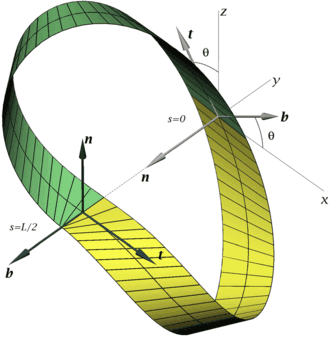Fig. 3

The conditions Eqs. (37)–(39) fix one end of the half strip at the origin and align the rotational symmetry axis with the y-axis. The conditions Eqs. (34)–(36) orient the Frenet–Serret frames at both ends as follows:

\begin{aligned} &\boldsymbol{t}(0) = \begin{pmatrix} -\sin\vartheta_0 \\ 0 \\ \cos\vartheta_0 \end{pmatrix}, \qquad \boldsymbol{n}(0) = \begin{pmatrix} 0 \\ -1 \\ 0 \end{pmatrix}, \qquad \boldsymbol{b}(0) = \begin{pmatrix} \cos\vartheta_0 \\ 0 \\ \sin\vartheta_0 \end{pmatrix} , \\ &\boldsymbol{t}(L/2) = \begin{pmatrix} 1 \\ 0 \\ 0 \end{pmatrix}, \qquad \boldsymbol{n}(L/2) = \begin{pmatrix} 0 \\ 0 \\ 1 \end{pmatrix}, \qquad \boldsymbol{b}(L/2) = \begin{pmatrix} 0 \\ -1 \\ 0 \end{pmatrix} , \end{aligned}

where ϑ 0=ϑ(0) is as yet unknown. It turns out that this choice of angles avoids the Euler-angle singularity at θ=0 in all our subsequent computations. Taken together these position and angle conditions eliminate all rigid-body degrees of freedom of the strip. We see that the tangent plane to the strip is orthogonal to the axis of symmetry at s=0 and contains this axis at s=L/2. Moreover, the principal normal n(0) points in the negative y-direction, as does the binormal b(L/2).

The left end condition Eq. (33) specifies the end point s=0 as a cylindrical point, while the right end condition Eq. (32) specifies the end point s=L/2 as a point with vanishing curvature (which together with the symmetry property means that it is an inflection point). Having the inflection point at the end of the interval prevents us from having to integrate through the inflection point. Note that the κ thus obtained is non-negative over the entire arclength interval. To maintain force and moment balance when assembling the entire strip we require that the components along the π-rotation symmetry axis vanish at s=0, hence Eqs. (30) and (31).

The final boundary condition comes from Eq. (21). Since we differentiated this equation to derive our system of ODEs, we need to impose Eq. (21) as a boundary condition to fix the integration constant. Using that η′(0)=0 and taking the limit lim s→0 κ g=2κ(0)(1+η 2(0))2, we may impose this condition in the form

$$M_3(0)=-\eta(0) M_1(0) - 2\kappa(0) \bigl(1+ \eta^2(0)\bigr)^2 .$$

Since the force vector is constant in space, Eq. (30) implies F 3(L/2)=0 because n(0)=b(L/2). Vanishing curvature and torsion at s=L/2 together with Eq. (21) entails M 3(L/2)=0. Note that F 3(L/2)=0 and M 3(L/2)=0 are in the fixed-point set of R 2. One can similarly verify that the boundary conditions cause an R 1 symmetric solution also to be R 2 symmetric. We could therefore also have used the R 2 involution about the inflection point at s=L/2 to obtain the full Möbius strip from the computed half strip.

Note that the boundary conditions do not include the condition η(L/2)=0. This condition is not required because it is enforced by symmetry: the boundary conditions at s=L/2 enforce a switching point at s=L/2 for the symmetric full strip (i.e., n(−L/2)=−n(L/2) and b(−L/2)=−b(L/2)) and according to the Randrup & Røgen properties of Sect. 5.1, at such points η=0, in addition to κ=0. For a nonsymmetric solution, η in fact need not be zero at switching points. The fact that we always find η tending to zero at s=L/2 (see below) is therefore evidence for the non-existence of nonsymmetric solutions. For the infinitely narrow strip, η in fact does approach a nonzero value, namely 1, at the switching point , which shows that there is no symmetric solution in that case.

The boundary-value problem is solved numerically by the continuation code AUTO . There are significant numerical difficulties solving this problem, as each end of the integration interval has a singularity. At the switching point enforced at s=L/2, |η′| is always found to tend to 1/w, giving a logarithmic singularity in Eq. (9) (this singular behaviour is consistent with analytical asymptotic results in ). In practice, to compute a starting solution, we first compute an approximate solution with κ(L/2)≈0.1 to stay away from the singularity at s=L/2. When all other boundary conditions are satisfied we ‘pull’ the solution into the singularity by continuing κ(L/2) to zero as far as possible, typically reaching values of 0.001. At this point, we typically have η(L/2) of the same order of magnitude while 1/w−|η′(L/2)|, the distance from the singularity, is typically as small as 10−6. At the other end (s=0), numerical convergence requires Taylor expansions of the coefficients a i , b i in Eqs. (25) about η′=0 to be used for a small interval around s=0 to eliminate the removable singularity of V in Eq. (9) (we use expansions up to fourth order). As a check on the numerical results, the first integrals |F| and FM are typically found to be constant to within 10−7.

Figures 4, 5 and 6 show numerically obtained solutions with $$Lk=\frac{1}{2}$$ (mirror images, having a link $$Lk=-\frac{1}{2}$$, are obtained by applying the reflection S). There is only one physical parameter in the problem, namely the aspect ratio L/(2w) of the strip. In the computations we have fixed L=2π and varied w. Also shown in the figures is the evolution along the strip of the straight generator. We note the points where the generators start to accumulate. At these points |′|→1 and the integrand in Eq. (8) (the energy density) diverges. Where this happens the generator rapidly sweeps through a nearly flat (violet) triangular region, a phenomenon readily observed in a paper Möbius strip (Fig. 1). We also observe two additional (milder) accumulations where no inflection occurs and the energy density remains finite. It can be shown that the energy density is monotonic along a generator. This implies that the (red) regions of high curvature cannot be connected by a generator, as a careful inspection confirms. Bounding the (violet) triangular (more precisely, trapezoidal) regions are two cylindrical generators of constant curvature (and hence constant colour in the figure) that realise local minima for the angle β.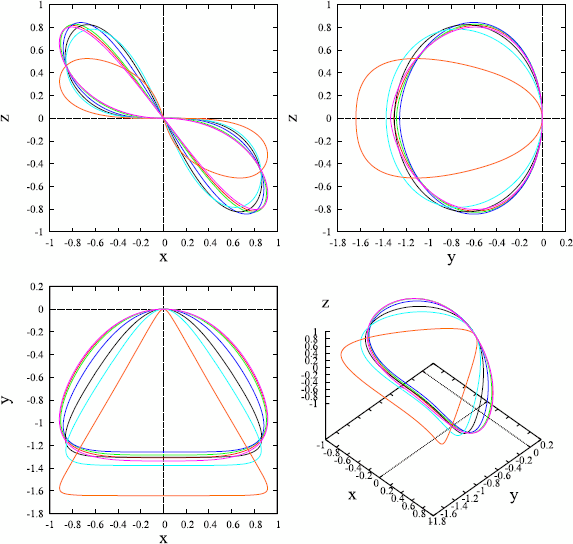Fig. 4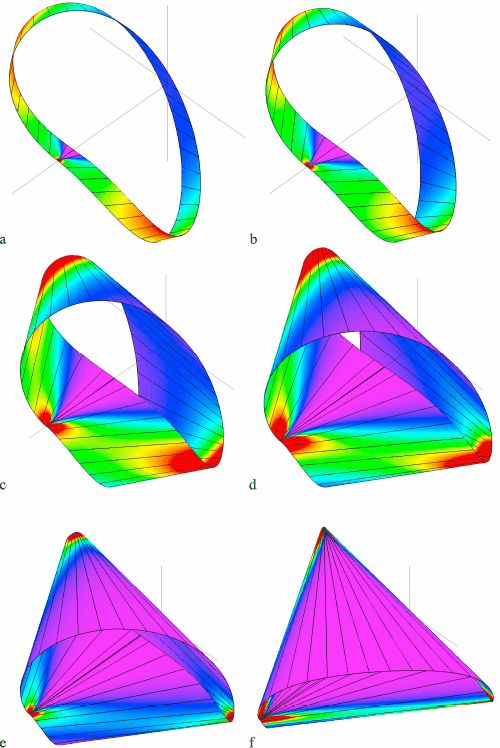Fig. 5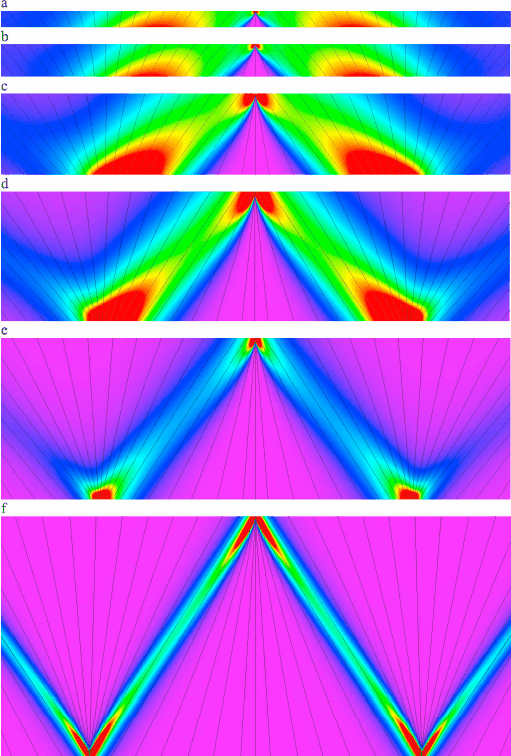Fig. 6

As w is increased the accumulations and associated triangular regions become more pronounced. At the critical value given by $$w=\pi/\sqrt{3}$$ the strip collapses into a triple-covered equilateral triangle [3, 48]. The folding process as w is increased towards this flat triangular limit resembles the tightening of tubular knots as they approach the ideal shape of minimum length to diameter ratio . In the flat limit the generators are divided into three groups, intersecting each other in three vertices. The bounding generators of constant curvature become the creases. It has been conjectured that a smooth developable Möbius strip can be isometrically embedded in $$\mathbb{R}^{3}$$ only if $$w<\pi/\sqrt{3}$$ , while it has been proven that a smooth developable Möbius strip can be immersed in $$\mathbb{R}^{3}$$ only if w<2 . Interestingly, smaller (in fact, arbitrarily small) values for the aspect ratio L/(2w) can be obtained if one allows for additional folding [3, 13].

Figures 7 and 8 give plots of curvature and torsion. The Randrup & Røgen property that κ=η=0 at an odd number of points is confirmed in Fig. 7 and can also be seen in Fig. 6 at the centre of the images where the generator makes an angle of 90 with the centreline. As the maximum value $$w_{c}=\pi/\sqrt{3}=1.8138\ldots$$ is approached, both curvature and torsion become increasingly peaked about s=0, 2π/3 and 4π/3. In the limit all bending and torsion is concentrated at the creases of the flat triangular shape. Going to the other extreme, we find that the solution in the limit of zero width has non-vanishing curvature, so that the Randrup & Røgen conditions are not satisfied. Given that the Frenet–Serret frame flips at s=π this means that the curvature is discontinuous. In addition, η tends to 1, giving a limiting generator angle β=45. Both these properties were anticipated in . This shows that the zero-width limit is singular and suggests that the Sadowsky problem has only a solution with discontinuous curvature.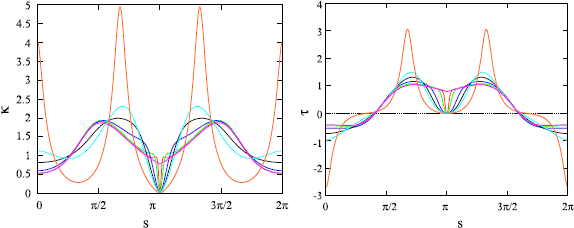Fig. 7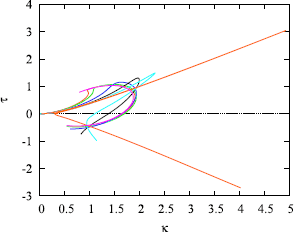Fig. 8

### Edge of Regression

The edge of regression for an equilibrium shape consists of three components (the red curves in Fig. 9). Each component has a cusp, corresponding to a swallow tail singularity of the asymptotic completion of the strip. This is the minimum number of singularities that a rectifying developable Möbius strip can have . Note that precisely one cusp lies on the edge of the strip at the umbilic generator (this is always observed, for all widths). The edge of regression goes to infinity when approaching a cylindrical point (having η′=0) and asymptotically tends to the direction of the generator at this point (see Fig. 10). Thus three cusps (corresponding to ‘conical’ points, having η″=0) alternate with three ‘cylindrical’ points.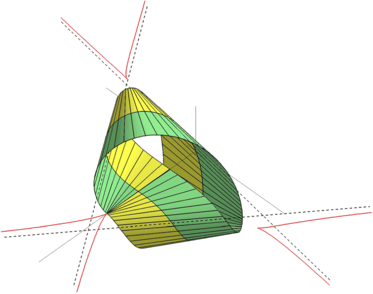Fig. 9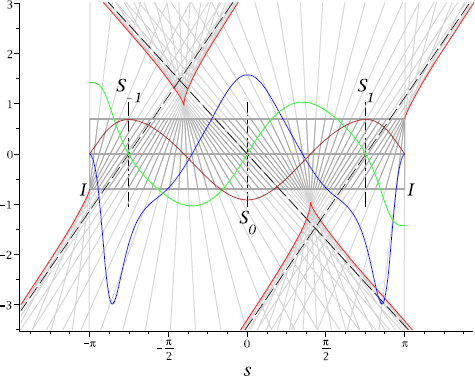Fig. 10

### Energy, Twist and Writhe of the Strip’s Centreline

The normalised energy $$\bar{U}=U/(Dw)$$ of the strip is shown in Fig. 11. The only meaningful energy estimate available in the literature, at the particular value of w=π/30, is that of Gravesen & Willatzen , who minimise $$\bar{U}$$ for the parametrised family of developable Möbius strips (not in equilibrium) constructed in . Their solution gives $$\bar{U}=9.22$$; at the same width we find the much lower value $$\bar{U}=5.93$$.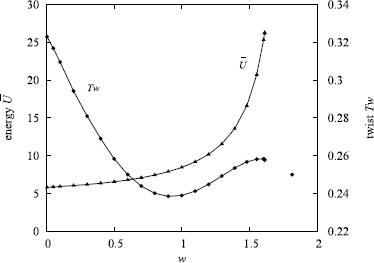Fig. 11

Also shown in Fig. 11 is the twist $$Tw=\frac{1}{2\pi}\int_{0}^{L}\tau\,\mathrm{d}s$$ of the strip as a function of w. Tw may be written as Tw=LkWr, where Lk is the linking number of the Möbius cord and Wr is the writhe of the centreline. Wr is commonly used as a measure for the spatial deformation of a 3D curve. It can be defined as the crossing number of the curve averaged over all viewing directions .

## Developable Strips of Higher Linking Number—Möbius Surgery

### Modified Boundary-Value Problem for D n -Symmetric Solutions

The Möbius strip defines only one example of a boundary-value problem for twisted sheets. A natural generalisation is to strips with linking numbers other than $$\pm\frac{1}{2}$$. The Randrup & Røgen properties of Sect. 5.1 also hold for these generalised Möbius strips and our techniques, exploiting symmetry, can readily be applied to such problems.

We have seen that the Möbius strip has π rotational symmetry about n (described by R 1) at the cylindrical point at s=0 (labelled S 0 in Fig. 10), where η′=0, and π rotational symmetry about b (described by R 2) at the inflection point at s=L/2 (labelled I in Fig. 10), where κ=0. Continuity of forces and moments across these points is ensured because the corresponding normal and binormal components vanish: F 2(0)=0=M 2(0) and F 3(L/2)=0=M 3(L/2). (Note that the rotational symmetry is also confirmed in Figs. 5 and 6 by the generators at these two points having constant colour, corresponding to the curvature being constant along these generators.)

We can therefore use involution R 1 at cylindrical points and involution R 2 at inflection points to construct solutions to the equilibrium equations with an arbitrary number of such points. In fact, the Möbius half strip has a second, intermediate, cylindrical point (S 1 in Fig. 10) with constant-colour generator. This point is not a point of full symmetry as F 2 and M 2 are nonzero. However, it turns out that by a slight change of orientation of the Frenet–Serret frame we can deform the strip such as to make these components zero. We then have a choice of taking either the (long) segment S 0 I or the (short) segment S 1 I as elementary piece in constructing more complicated equilibrium shapes. Note from Fig. 10 that one difference between these two pieces is that S 1 I has positive η (and hence positive torsion τ), while on S 0 I η (and hence τ) changes sign. The shapes constructed by combining these pieces will in general not be closed. However, by imposing symmetric closure conditions we can obtain D n -symmetric solutions for n=2,3,4,5,… .

We thus reformulate the boundary-value problem as follows. We drop the two frame orientation conditions at s=0 in the previous set of boundary conditions in Sect. 5.2 and specify, on the interval [0,L/(2n)] (see Fig. 12),

\begin{aligned} F_2(0) =& 0,\qquad\ F_3\bigl(L/(2n)\bigr) =0 , \end{aligned}
(40)
\begin{aligned} M_2(0) =& 0,\qquad M_3\bigl(L/(2n)\bigr) =0 , \end{aligned}
(41)
\begin{aligned} & \phantom{\qquad\quad\ \ \,} \kappa\bigl(L/(2n)\bigr) = 0, \end{aligned}
(42)
\begin{aligned} \eta'(0) =& 0, \end{aligned}
(43)
\begin{aligned} & \phantom{\qquad\quad\ \,\,} \psi\bigl(L/(2n)\bigr) = 2\pi, \end{aligned}
(44)
\begin{aligned} & \phantom{\qquad\quad\ \ \,} \vartheta\bigl(L/(2n)\bigr) = \frac{\pi}{2}, \end{aligned}
(45)
\begin{aligned} & \phantom{\qquad\quad\ \ \,} \phi\bigl(L/(2n)\bigr) = \pi, \end{aligned}
(46)
\begin{aligned} & \phantom{\qquad\quad\ \ \,} x\bigl(L/(2n)\bigr) =0, \end{aligned}
(47)
\begin{aligned} & \phantom{\qquad\quad\ \,\,} y\bigl(L/(2n)\bigr) = 0, \end{aligned}
(48)
\begin{aligned} & \phantom{\qquad\quad\ \,\,\,} z\bigl(L/(2n)\bigr) = 0. \end{aligned}
(49)

These boundary conditions ‘cut out’ a piece between cylindrical and inflection points (either S 0 I or S 1 I) of the Möbius strip solution.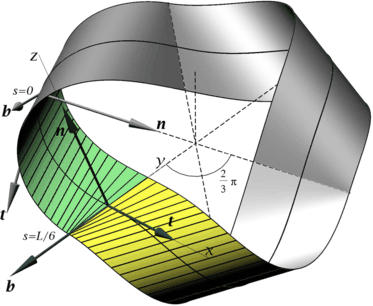Fig. 12

We then add two symmetric closure conditions for a solution consisting of a sequence of 2n congruent pieces satisfying the above boundary conditions. The first condition enforces coplanarity of n(0), b(L/(2n)) and r(L/(2n))−r(0):

$$\bigl(\boldsymbol{n}(0) \times \boldsymbol{b}\bigl(L/(2n)\bigr)\bigr) \cdot \bigl(\boldsymbol{r} \bigl(L/(2n)\bigr)-\boldsymbol{r}(0)\bigr) = z(0) n_x(0) - x(0) n_z(0)=0.$$
(50)

In explicit form, after using the boundary conditions (40)–(49), this reads

$$z(0) \bigl(-\sin\psi(0) \sin\varphi(0)+\cos\vartheta(0) \cos\psi(0) \cos \varphi(0) \bigr) + x(0) \sin\vartheta(0) \cos\varphi(0)=0.$$
(51)

The second condition enforces an angle $$\frac{m}{n}\pi$$, $$\frac{m}{n} \in \mathbb{Q} \setminus \mathbb{Z}$$, m<n, between n(0) and b(L/(2n)):

$$\boldsymbol{n}(0) \cdot \boldsymbol{b}\bigl(L/(2n)\bigr) = \cos \biggl(m \frac{\pi}{n} \biggr) .$$
(52)

This condition guarantees that concatenation of 2n copies of the elementary piece produces a closed strip (with n inflection points). In our variables the condition reads

$$-\cos\psi(0) \sin\varphi(0) -\cos\vartheta(0) \sin\psi(0) \cos\varphi(0) = \cos \biggl(m \frac{\pi}{n} \biggr) .$$
(53)

The D n -symmetry axis is directed along the vector (z(0),0,−x(0)) and intersects the y-axis at

\begin{aligned} y_c =& \biggl[ \biggl(\boldsymbol{b}\bigl(L/(2n)\bigr)-\boldsymbol{n}(0) \cos \biggl(m \frac{\pi}{n} \biggr) \biggr) \cdot \bigl(\boldsymbol{r}\bigl(L/(2n) \bigr)-\boldsymbol{r}(0)\bigr) \biggr] \csc^2 \biggl(m \frac{\pi}{n} \biggr) \\ =&\bigl(\boldsymbol{r}\bigl(L/(2n)\bigr)-\boldsymbol{r}(0)\bigr) \cdot \boldsymbol{b}\bigl(L/(2n) \bigr) \\ &{}-\sqrt{\bigl(\boldsymbol{r}\bigl(L/(2n)\bigr)-\boldsymbol{r}(0)\bigr)^2-\bigl( \bigl(\boldsymbol{r}\bigl(L/(2n)\bigr)-\boldsymbol{r}(0)\bigr) \cdot \boldsymbol{b}\bigl(L/(2n)\bigr) \bigr)^2} \cot \biggl(m \frac{\pi}{n} \biggr) \\ =&y(0)-\sqrt{x^2(0)+z^2(0)}\cot \biggl(m \frac{\pi}{n} \biggr) . \end{aligned}

Note that the C 2-symmetric Möbius strip solution of Sect. 5, having (m,n)=(0,1), is degenerate for these boundary conditions as the first symmetry condition, Eq. (51), is identically satisfied since x(0)=z(0)=0.

### Two-Sided Strip with (m,n)=(1,2)—Figure-of-Eight (Lk=1)

For (m,n)=(1,2) we have a two-sided solution with Lk=1 in the shape of a figure-of-eight. A simple instance of this solution is a cylindrical shape with planar centreline and with all generators orthogonal to this centreline (η≡0). This is the figure-of-eight elastica solution with self-intersection . A non-selfintersecting solution may be obtained by considering a slightly displaced solution with non-planar centreline and allowing for self-contact (see Fig. 13). For a D 2-symmetric solution we assume, prompted by paper models, that this self-contact occurs at the inflection point. If we then also assume that the contact at the edge of the strip is frictionless then the contact force will only have a component in the binormal direction, i.e., along the umbilical generator through the inflection point. We can compute such solutions by dropping the second boundary condition in Eq. (40) and imposing the geometrical contact condition y c =w, which now becomes y(0)=w, instead. The end force F 3(L/4) is then allowed to become nonzero. For a physically realisable solution we require F 3(L/4)<0 so that it is balanced by an equal and opposite contact force in the (positive) binormal direction coming from the other half of the strip (see Fig. 13).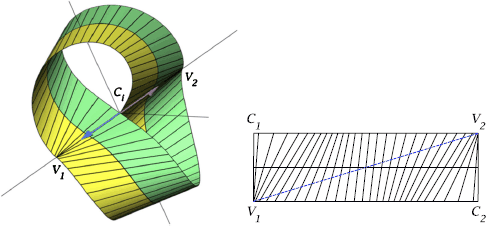Fig. 13

Three examples of strips with self-contacts, constructed from (short) S 1 I pieces of the Möbius strip, are shown in Fig. 14. The solutions have three cylindrical points between the two inflection points, which means that in deforming the S 1 I piece to satisfy the symmetric closure conditions an extra intermediate cylindrical point has appeared. The solutions in Fig. 14(a, b) have, however, retained the property of S 1 I that they have positive torsion, while those in (c) and (d) have slightly negative torsion (in the top and bottom violet regions in the figure).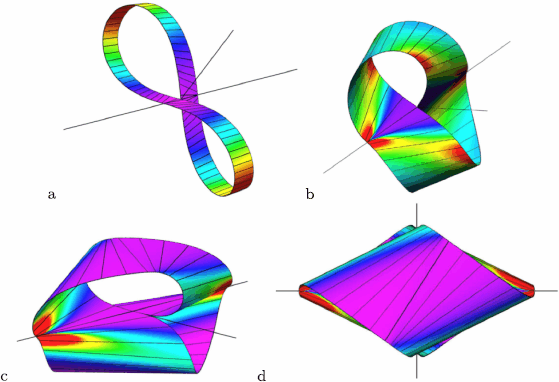Fig. 14

Dependence of the contact force 2F 3(L/4) on the aspect ratio of the strip is plotted in Fig. 15. The contact force vanishes only in the zero-width limit w=0. This seems to suggest that no finite-width solutions exist that are free of self-contact. However, there may be different types of (m,n)=(1,2) solutions with no self-contact and indeed Fig. 16 below gives an example of exactly such a solution.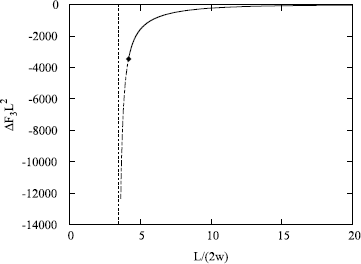Fig. 15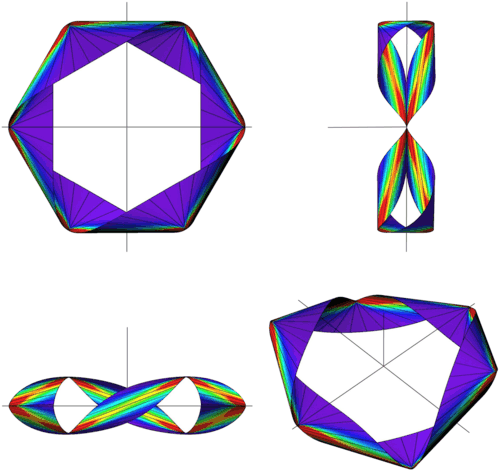Fig. 16

Figure 14 shows that as the width w increases the figure-of-eight increasingly flattens. The aspect ratio of the strip is bounded from below. To see this note from Fig. 13 (left) that the points V 1 and V 2 of the umbilic generators lying on the outer edges opposite to the contact points are separated by twice the width of the strip: |V 1 V 2|=4w. This means that in the planar development of the strip the straight line connecting V 1 and V 2 cannot be shorter than 4w (Fig. 13, right), so we conclude that the length of the centreline cannot be less than $$4w\sqrt{3}$$ and the aspect ratio L/(2w) cannot go below $$2\sqrt{3}$$ (consistent with the asymptotic behaviour displayed in Fig. 15).

If we imagine continuation of the sequence in Fig. 14 to a completely flattened triple-covered rhombus, with diagonals of length 4w and $$4w/\sqrt{3}$$, then this solution would have exactly the limiting aspect ratio. (By allowing other such sharp folds we can in fact reach strips of the same topology with lower aspect ratio.)

However, numerical results show that, for our equilibrium solutions, the strip starts to self-intersect near the contact point before this flat state is reached. This indicates that shapes with a single contact do not exist beyond a critical value of w. Shapes with multiple contact points are beyond the scope of the present work, but it appears that the contact point bifurcates giving rise to other, nonsymmetric, solutions. The onset of this self-intersection coincides with the tangents to the edges at the contact point becoming aligned with each other (at the diamond in Fig. 15). This tangent alignment happens when t(L/2) becomes parallel to the rotational symmetry axis, which can be represented as the vector n(0)×b(L/2). Hence the condition is t(L/2)×(n(0)×b(L/2))=0, which simplifies to n(0)⋅t(L/2)=0 and further to x(0)=0. In our continuation run this condition is met at aspect ratio L/(2w)=4.218. Figures 14(c, d) show the solution at this value.

We finally show, in Fig. 16, an example of a (m,n)=(1,2) strip with Lk=1 constructed from two (long) S 0 I pieces of the Möbius strip. The solution is not self-contacting and has the additional cylindrical points between inflection points from the S 0 I piece. The number of cylindrical and conical points is therefore the same as that of the solutions in Fig. 14. At the four conical points η and κ are small and in this sense the conical points are close to being inflection points; they share with the two inflection points the features of radiating sharp ridges that bound flat triangular regions (similar to the Möbius strip solutions in Figs. 5 and 6). In projection the solution has almost perfect D 6 symmetry. Despite the vastly different appearances, the main difference between the solutions in Fig. 14(a, b) and Fig. 16 is that the former have all positive torsion while the latter has a torsion that changes sign (at non-inflection points where the generator is perpendicular to the centreline).

### One-Sided Strip with (m,n)=(2,3)—Strip with Lk=3/2

For (m,n)=(2,3), taking three copies of a S 1 I piece of the Möbius strip, we get a one-sided strip with $$Lk=\frac{3}{2}$$ (also known from Escher’s work ). Three examples at different aspect ratios are shown in Fig. 17, with the last (c) showing the shape at initial (triple) self-contact at the central axis, when y c =w. The development of one third of this shape is shown in Fig. 18. The points C 1 and C 2 coincide in space at the point of triple contact. All three solutions have positive torsion and, unlike for the figure-of-eight solutions in Fig. 14, no intermediate cylindrical points have appeared in the deformation of the S 1 I piece.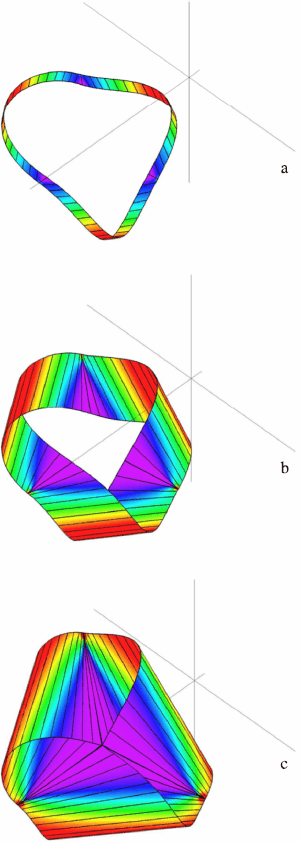Fig. 17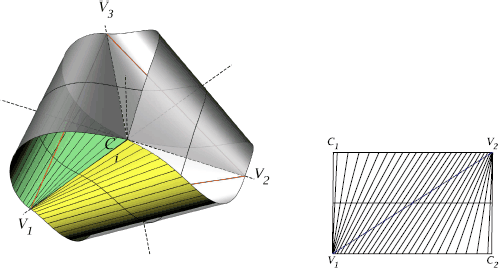Fig. 18

D 3-symmetric solutions for wider strips, without self-intersection, can be obtained by imposing an impenetrability constraint and introducing a contact force. Thus we assume that the single triple contact point is maintained by three contact forces of equal magnitude acting along the respective umbilic binormals (the lines C i V i ,i=1,2,3, in Fig. 18). The contact forces are then coplanar and therefore self-balancing (note that these forces applied at the edge of the strip produce no moments when transferred to the centreline of the strip).

The boundary-value problem is then modified as in the previous section for the figure-of-eight solution by dropping the second condition in Eq. (40) and adding the geometrical contact condition y c =w instead. The end force F 3(L/6) is thus allowed to become nonzero. An example of such a self-contacting solution is shown in Fig. 19.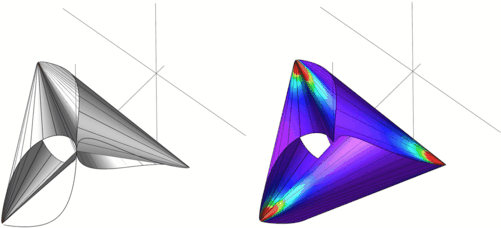Fig. 19

There is again a lower bound to the aspect ratio of the strip. The three coplanar binormals C i V i make an equilateral triangle V 1 V 2 V 3 with sides $$2 w \sqrt{3}$$ (see Fig. 18) and the distance V 1 V 2 measured on the strip’s surface cannot be shorter than $$2 w \sqrt{3}$$, this minimum value occurring only if the entire straight line connecting V 1 and V 2 lies in the surface. This minimum value for $$|V_{1} V_{2}|=2 w \sqrt{3}$$ then gives the minimum aspect ratio $$L/(2w)= 3\sqrt{2}$$. In this limit, V 1 V 2 becomes a generator and all other generators pass through the points V i . The surface is thus conical. One can also show that η becomes a piecewise linear function of arclength: $$\eta=\sqrt{2} (|4\{\sqrt{2} s/(8w)\}-2|-1)$$, $$s \in [0,6\sqrt{2}w]$$, where {x} denotes the fractional part of x. Figure 20 shows how η approaches this conical limit. Looking at Fig. 19 one can indeed imagine a limiting shape consisting of three conical surfaces with their apices at V 1, V 2 and V 3. The conical patches join each other along the three generators V 1 V 2, V 2 V 3 and V 3 V 1. We study the conical limit of our equations further in Appendix B.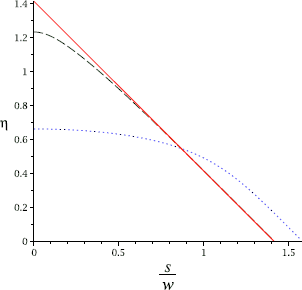Fig. 20

The combined tri-conical surface can be made arbitrarily smooth (by appropriate local change of the curvature). The resulting surface then provides an interesting example of a developable surface that is arbitrarily smooth yet does not have a smooth (just a continuous) field of generators (since η(s) lacks differentiability at points where the centreline crosses either of the three generators V 1 V 2, V 2 V 3 or V 3 V 1) [43, 57].

Returning to equilibrium solutions, our computational results suggest that just before the limiting aspect ratio, at L/(2w)=4.249, all three tangents to the edges in the central triple contact point C i become aligned along the D 3-symmetry axis. This solution is shown in Fig. 19. The strip has no other contacts and does not self-intersect. However, as in the case of the figure-of-eight solution, this alignment of tangents appears to be the onset of self-intersection. To find the limiting shape of a physical strip we would therefore have to account for contact more complicated than single-point contact, a problem we leave for future work.

Experimentation with paper models also suggests that at or shortly after the first contact a pitchfork bifurcation occurs where the D 3-symmetric solution becomes unstable and two stable solutions appear, buckled in opposite directions. For such nonsymmetric solutions the three contacting umbilic generators would no longer be coplanar. They may or may not continue to be perpendicular to the edge of the strip (η=0). If they are then there would only be a normal component in addition to the binormal component of the contact force at the edge of the strip, and hence at the centreline. This edge component would induce a twisting moment M 1 at the centreline that would also have to be taken into account. If the umbilic generators are no longer perpendicular to the edge then all force and moment components of the contact force would have to be accounted for. In the wide limit we can imagine a folded shape in the form of a tetrahedron with three faces being double-covered right triangles with sides 2w, 2w and $$2w\sqrt{2}$$, while the fourth face is absent. It is easy to see that such a ‘quarter-cube’ configuration, with residual C 3-symmetry, has aspect ratio L/(2w)=3.

In Fig. 21 we finally show an example of a (m,n)=(2,3) strip with Lk=3/2 constructed from three (long) S 0 I pieces of the Möbius strip. The solution is not self-contacting. It follows the pattern of the Lk=1 solution in Fig. 16 and in projection has approximate D 9-symmetry.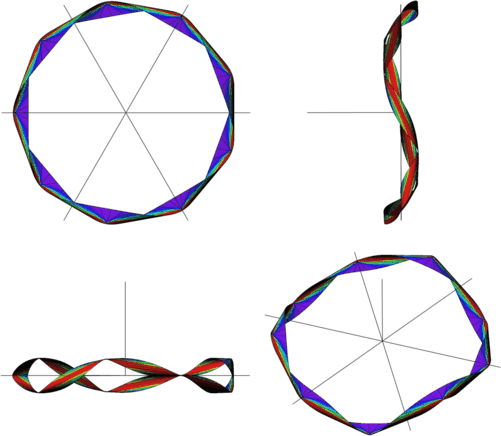Fig. 21

### Higher-Order One- and Two-Sided Developable Strips

The series of closed-strip solutions may be continued by increasing the number of congruent pieces. Figure 22 shows strips made of 6, 8, 10 and 12 pieces of type S 1 I for, respectively, m=2,3,4,5 and n=m+1. By construction each strip possesses the corresponding D n -symmetry. The edges of the strips form torus knots or links of type (n,2). The centrelines are unknotted. Each of these solutions has n isolated cylindrical points, the same as the number of inflection points. Solutions are alternatingly one- or two-sided. As the width is increased the strips become flatter (as illustrated by the bottom figures in side projection), tending to flat folded shapes with the inflection points at vertices of regular n-gons. One easily verifies that these limiting flat shapes have aspect ratio $$L/(2w) = n \cot \frac{\pi}{n}$$, n=3,4,5,…. The strip for n=3, that we also discussed in the previous section, has self-contact before its width reaches this critical value, while for n=4 self-contact coincides with the final shape, which is a double-covered square of side $$2\sqrt{2}w$$ (equal to the distance V i V i+1 between singularities on the edge of the strip) and centreline length L=8w. Solutions for n>4 do not have self-contacts. We cannot rule out that D n -symmetric shapes with multiple central contact exist at higher aspect ratio, but we have not found such elastic strips in equilibrium.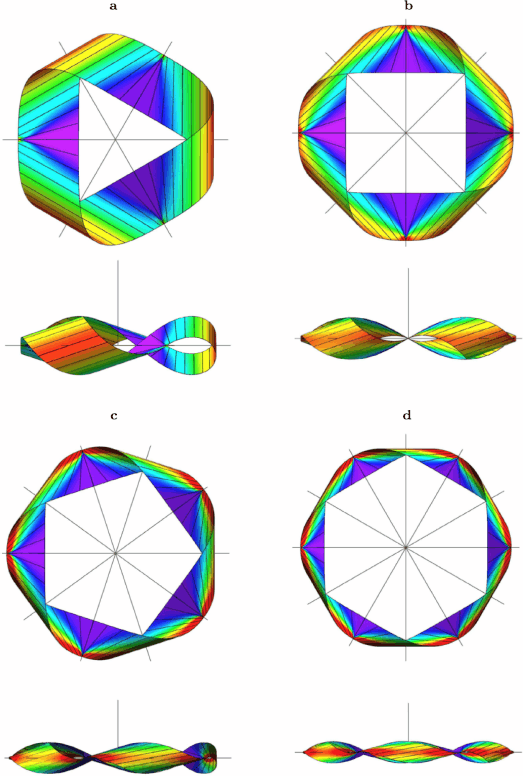Fig. 22

Figure 23 shows a series of D n -symmetric solutions with 2n (n=3,4,5,6) congruent pieces of type S 0 I with an extra cylindrical point in the interior. These shapes therefore have 3n cylindrical points 2n of which generically do not lie on a C 2-symmetry axis (the number of inflection points remains n). In general, computed solutions may easily have self-intersections (or undergo self-crossings during parameter continuation, which affect the linking number), particularly at smaller aspect ratios, but all presented solutions are non-selfintersecting.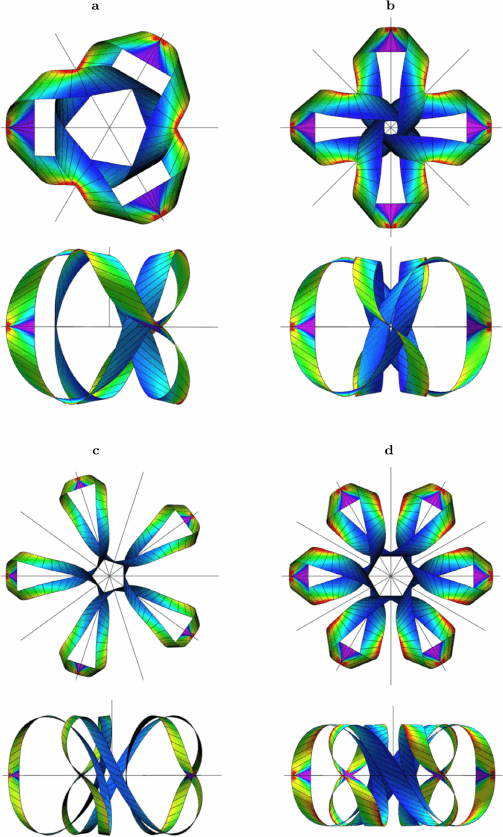Fig. 23

The final two shapes in Fig. 24, for (m,n)=(2,5) and (3,5), complement the set of D 5-symmetric solutions made of 10 pieces (the (m,n)=(4,5) and (1,5) solutions are shown in Figs. 22 and 23, respectively). Each has 5 inflection points and the left strip has 15 cylindrical points while the right one has only 5.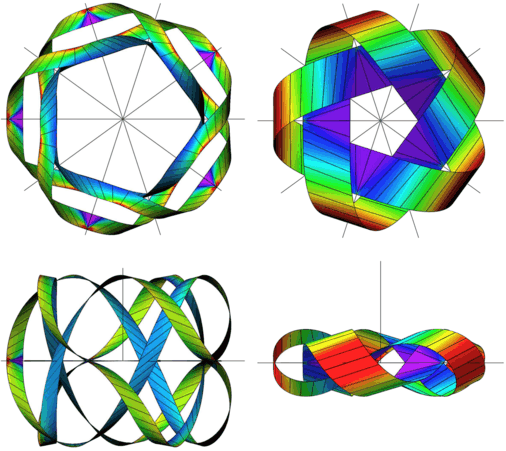Fig. 24

## Discussion

We have formulated and numerically solved boundary-value problems for the isometric deformation of flat elastic sheets. Both one- and two-sided topologies have been considered. A common feature in all computed solutions is the existence of singularities of infinite bending energy density on the edge of the strip. Symmetry was used to construct solutions with multiple edge singularities from elementary pieces of the Möbius strip with a single singularity. This technique can be applied to find equilibrium shapes with any number of inflection points. Nonsymmetric solutions could be obtained by solving multiple boundary-value problems with different end conditions but with matching conditions at interior points. Our approach, of course, applies to open strips as well as to closed strips. In  we used a technique similar to the Möbius surgery discussed here to construct triangular buckling patterns of twisted strip in good agreement with experimental observations.

We used the developability property to reduce the energy integral to a single integral over the centreline thus obtaining a one-dimensional variational problem. The great advantage of this reduction is that the Euler–Lagrange equations for this problem are ODEs rather than the usual PDEs of plate theory. The relatively small price we pay is that the reduced variational problem is second-order but efficient equilibrium equations, Eqs. (16), (17), (21), (22), were derived in Euler–Poincaré form. A similar approach was taken in  to derive equilibrium equations for braided rods.

This reduction generalises to more general strips with prescribed boundaries in the intrinsic geometry, although it may be necessary to choose a non-straight centreline (reference curve) to reduce to (κ g ≠0). In this case determining the bounds of the t-integration in Eq. (7) may require the solution of algebraic equations, which may render the reduction impractical. For simple geometries, however, for instance for an annular strip, reduction, at least in the narrow limit, is straigthforward .

Another generalisation is to strips that are developable but not necessarily planar in their relaxed state. Since we use the parametrisation of a developable surface in Eq. (1), the short edges of the strip must be generators in the deformed state. Subject to this constraint on the deformation, generalisations are possible (see Appendix A for more on this). For instance, for a strip whose relaxed surface belongs to a cylinder (i.e., η=const.), the reduction to the centreline can still be carried out (see  for an example). Extension to strips with anisotropic elasticity is straightforward.

The Möbius strip is usually treated as a topological or a geometrical object, not a mechanical one. One of the few previous attempts to compute equilibrium shapes of a Möbius strip was by Mahadevan & Keller  who employed a thin anisotropic elastic rod model. They obtained asymptotic equations for large values of the aspect ratio of the rod’s cross-section. This limit corresponds to perfect alignment of the rod material frame and the Frenet–Serret frame, and the equilibrium equations are therefore the Euler–Lagrange equations for Lagrangian $${\bar{l}}=\kappa^{2}(1+\frac{C}{B}\eta^{2})$$ in Eq. (12), where B and C are the bending and torsional stiffnesses, respectively. This corresponds to a strip formed by the binormal as it moves along the rod’s centreline. The solution to those equations, however, although closed as a rod, does not describe a closed strip, even after the modifications made by the authors. In particular, it does not satisfy the Randrup & Røgen conditions of Sect. 5.1, and therefore cannot serve as the centreline of a developable Möbius strip, not even a narrow one. It does not capture the essential geometrical properties of such a strip, for instance the fact that the torsion τ is zero in the inflection point (required for the generators to line up).

The geometrical features and accompanying stress localisation of Möbius and other developable strips observed here are seen more widely in problems of elastic sheets such as paper folding or crumpling and fabric draping, and were already noted in . Crumpling of paper is dominated by bending along ridges bounding almost flat regions or facets [31, 58], behaviour that we see back in the nearly flat triangular regions in Fig. 5. In fabric draping, triangular regions are seen to form that radiate out from (approximate) vertices . The formation of these flat triangular regions appears to be a generic feature of nature’s response to twisting inextensible sheets. Analytical work on such sheets often assumes regions of localisation of bending energy in the form of vertices of conical surfaces [5, 6, 18]. It is known that conical surfaces have infinite elastic energy within the linear elastic theory. A cut-off near the cone apex was therefore introduced in  to obtain the equilibrium equations. More recently, local constraints on the surface metric have been incorporated into a variational framework to describe equilibria of generalised conical surfaces made of unstretchable flat sheets [18, 19]. As the examples in our present work show, by using non-conical developable elastic surfaces one can describe bending localisation phenomena without the need for a cut-off.

Importantly, our approach predicts the emergence of regions of high bending. Although we prescribe the number of inflection (switching) points of the solution we never impose any a priori constraints on the shape of the strip, neither in the vicinity of the inflection points nor elsewhere. Points of divergence of the bending energy may serve as indicators of positions where out-of-plane tearing (fracture failure mode III) is likely to be initiated. In this respect it is interesting to observe that when one tries to tear a piece of paper one intuitively applies a torsion, thereby creating intersecting creases as in the vertices of the central triangular domains in Fig. 5. A crack originates at the vertex, where the energy density diverges.

Curvature singularities are of interest to studies of twisted graphene-based nanoribbons. All-atom first-principle simulations of zigzag-edged graphene nanoribbons in the form of a Möbius strip show a shape evolution under varying aspect ratio that is in good agreement with our results in Fig. 5 . Other simulation results reveal that the local curvature of nanoribbons increases at defect sites . A seemingly related ‘twist localisation’ was observed in ab initio calculations of twisted cyclacenes .

Recent technological developments, such as nanostructured origami  and strain engineering of nanomembranes , have demonstrated the possibility of producing extremely thin membrane structures that can be folded in a controlled fashion. These structures have great potential as components of electromechanical devices such as force probes, capacitors, resonators, etc. with potentially unusual physical properties. For instance, silicon nanomembranes (SiNMs), consisting of the same material as bulk Si-based semiconductors, have been shown to become electrical conductors when the membrane is sufficiently thin. There is, therefore, significant interest in the relationship between geometry (and topology) and transport and optical properties such as electrical conductance and photoluminescence. In  it was shown that in thin conducting sheets electrons increasingly localise to the high-curvature regions as the sheet folds, with creases forming channels for electron transport. Our methods presented in this paper may be used to find how thin free-standing sheets fold and where creases form.

1. 1.

Manufacturing an accurate model of an elastic Möbius strip is not entirely straightforward. If we glue the ends together with a small region of overlap then the resulting model will have non-uniform thickness, which affects the bending stiffness locally and hence the equilibrium shape in space. Welding the ends of a metal or plastic strip would have similar effects. To overcome these problems the model shown in Fig. 1 was created by printing the computed solution on a strip of double the length and then closing it after winding it twice, thus creating a strip of double the thickness. The two layers were then glued together without the need for an overlap and the thickness of the resulting strip is constant everywhere. The colouring was carefully phased in accordance with the actual shape.

2. 2.

Throughout we adopt the notation that for any vector $$\boldsymbol{v} \in \mathbb{R}^{3}$$ the sans-serif symbol v denotes the triple of components (v 1,v 2,v 3)=(vt,vn,vb) in the Frenet–Serret frame.

## References

1. 1.

Arora, W.J., Nichol, A.J., Smith, H.I., Barbastathis, G.: Membrane folding to achieve three-dimensional nanostructures: nanopatterned silicon nitride folded with stressed chromium hinges. Appl. Phys. Lett. 88(5), 053108 (2006). doi:10.1063/1.2168516

2. 2.

Ashwell, D.G.: The inextensional twisting of a rectangular plate. Q. J. Mech. Appl. Math. 15(1), 91–107 (1962). doi:10.1093/qjmam/15.1.91

3. 3.

Barr, S.: Experiments in Topology. Thomas Y. Crowell Company, New York (1964)

4. 4.

Caetano, E.W.S., Freire, V.N., dos Santos, S.G., Albuquerque, E.L., Galvão, D.S., Sato, F.: Defects in graphene-based twisted nanoribbons: structural, electronic, and optical properties. Langmuir 25(8), 4751–4759 (2009). doi:10.1021/la803929f

5. 5.

Cerda, E., Chaieb, S., Melo, F., Mahadevan, L.: Conical dislocations in crumpling. Nature 401, 46–49 (1999). doi:10.1038/43395

6. 6.

Cerda, E., Mahadevan, L., Pasini, J.M.: The elements of draping. Proc. Natl. Acad. Sci. USA 101(7), 1806–1810 (2004). doi:10.1073/pnas.0307160101

7. 7.

Chicone, C., Kalton, N.J.: Flat embeddings of the Möbius strip in R 3. Commun. Appl. Nonlinear Anal. 9, 31–50 (2002)

8. 8.

Chubelaschwili, D., Pinkall, U.: Elastic strips. Manuscr. Math. 133(3–4), 307–326 (2010). doi:10.1007/s00229-010-0369-x

9. 9.

Dias, M.A., Dudte, L.H., Mahadevan, L., Santangelo, C.D.: Geometric mechanics of curved crease origami. Phys. Rev. Lett. 109, 114301 (2012). doi:10.1103/PhysRevLett.109.114301

10. 10.

Doedel, E., et al.: AUTO: software for continuation and bifurcation problems in ordinary differential equations. In: User’s Manual, Concordia University, Montreal, Canada (2007). http://indy.cs.concordia.ca/auto/

11. 11.

Emmer, M.: Visual art and mathematics: the Moebius band. Leonardo 13(2), 108–111 (1980)

12. 12.

Friesecke, G., James, R.D., Mora, M.G., Müller, S.: Derivation of nonlinear bending theory for shells from three-dimensional nonlinear elasticity by gamma-convergence. C. R. Math. 336, 697–702 (2003). doi:10.1016/S1631-073X(03)00028-1

13. 13.

Fuchs, D., Tabachnikov, S.: Mathematical Omnibus: Thirty Lectures on Classic Mathematics. American Mathematical Society, Providence (2007)

14. 14.

Fuller, F.B.: The writhing number of a space curve. Proc. Natl. Acad. Sci. USA 68(4), 815–819 (1971)

15. 15.

Fuller, F.B.: Decomposition of the linking number of a closed ribbon: a problem from molecular biology. Proc. Natl. Acad. Sci. USA 75(8), 3557–3561 (1978)

16. 16.

Gay-Balmaz, F., Holm, D.D., Meier, D.M., Ratiu, T.S., Vialard, F.X.: Invariant higher-order variational problems. Commun. Math. Phys. 309, 413–458 (2012). doi:10.1007/s00220-011-1313-y

17. 17.

Gravesen, J., Willatzen, M.: Eigenstates of Möbius nanostructures including curvature effects. Phys. Rev. A 72, 032108 (2005). doi:10.1103/PhysRevA.72.032108

18. 18.

Guven, J., Müller, M.M.: How paper folds: bending with local constraints. J. Phys. A, Math. Theor. 41(5), 055203 (2008). doi:10.1088/1751-8113/41/5/055203

19. 19.

Guven, J., Müller, M.M., Vázquez-Montejo, P.: Conical instabilities on paper. J. Phys. A, Math. Theor. 45(1), 015203 (2012). doi:10.1088/1751-8113/45/1/015203

20. 20.

Halpern, B., Weaver, C.: Inverting a cylinder through isometric immersions and isometric embeddings. Trans. Am. Math. Soc. 230, 41–70 (1977). http://links.jstor.org/sici?sici=0002-9947%28197706%29230%3C41%3AIACTII%3E2.0.C0%3b2-I

21. 21.

Hangan, T.: Elastic strips and differential geometry. Rend. Semin. Mat. (Torino) 63(2), 179–186 (2005)

22. 22.

Hangan, T., Murea, C.: Elastic helices. Rev. Roum. Math. Pures Appl. 50(5-6), 641–645 (2005)

23. 23.

Hayashi, M., Ebisawa, H.: Little-parks oscillation of superconducting Möbius strip. J. Phys. Soc. Jpn. 70(12), 3495–3498 (2001)

24. 24.

Hornung, P.: Euler–Lagrange equations for variational problems on space curves. Phys. Rev. E 81(6), 066603 (2010). doi:10.1103/PhysRevE.81.066603

25. 25.

Hornung, P.: Euler–Lagrange equation and regularity for flat minimizers of the Willmore functional. Commun. Pure Appl. Math. 64(3), 367–441 (2011). doi:10.1002/cpa.20342

26. 26.

Kirby, N., Fried, E.: Gamma-limit of a model for the elastic energy of an inextensible ribbon (2013). arXiv:1307.3540 [math.AP]. 10 p.

27. 27.

Korte, A.P., van der Heijden, G.H.M.: Curvature-induced electron localization in developable Möbius-like nanostructures. J. Phys. Condens. Matter 21(49), 495301 (2009). doi:10.1088/0953-8984/21/49/495301

28. 28.

Korte, A.P., Starostin, E.L., van der Heijden, G.H.M.: Triangular buckling patterns of twisted inextensible strips. Proc. R. Soc. Lond. Ser. A 467(2125), 285–303 (2010). doi:10.1098/rspa.2010.0200

29. 29.

Kurono, Y., Umehara, M.: Flat Möbius strips of given isotopy type in $$\mathbb{R}^{3}$$ whose centerlines are geodesics or lines of curvature. Geom. Dedic. 134(1), 109–130 (2008). doi:10.1007/s10711-008-9248-y

30. 30.

Lagally, M.G.: Strain engineered silicon nanomembranes. J. Phys. Conf. Ser. 61(1), 652–657 (2007). doi:10.1088/1742-6596/61/1/131

31. 31.

Lobkovsky, A., Gentges, S., Li, H., Morse, D., Witten, T.A.: Scaling properties of stretching ridges in a crumpled elastic sheet. Science 270(5241), 1482–1485 (1995). doi:10.1126/science.270.5241.1482

32. 32.

Love, A.E.H.: A Treatise on the Mathematical Theory of Elasticity Dover, New York (1944), reprinted. Cambridge University Press (1927)

33. 33.

Mahadevan, L., Keller, J.B.: The shape of a Möbius band. Proc. R. Soc. Lond. Ser. A 440, 149–162 (1993)

34. 34.

Mansfield, E.H.: The inextensional theory for thin flat plates. Q. J. Mech. Appl. Math. 8(3), 338–352 (1955). doi:10.1093/qjmam/8.3.338

35. 35.

Mansfield, E.H.: The Bending and Stretching of Plates, 2nd edn. Cambridge University Press, Cambridge (1989)

36. 36.

Martín-Santamaría, S., Rzepa, H.S.: Twist localisation in single, double and triple twisted Möbius cyclacenes. J. Chem. Soc., Perkin Trans. 2, pp. 2378–2381 (2000). doi:10.1039/B005560N

37. 37.

Naokawa, K.: Singularities of the asymptotic completion of developable Möbius strips. Osaka J. Math. 50(2), 425–437 (2013)

38. 38.

Neff, P.: A geometrically exact Cosserat shell-model including size effects, avoiding degeneracy in the thin shell limit. Part I: Formal dimensional reduction for elastic plates and existence of minimizers for positive Cosserat couple modulus. Contin. Mech. Thermodyn. 16(6), 577–628 (2004). doi:10.1007/s00161-004-0182-4

39. 39.

Randrup, T., Røgen, P.: Sides of the Möbius strip. Arch. Math. 66(6), 511–521 (1996). doi:10.1007/BF01268871

40. 40.

Røgen, P.: Embedding and knotting of flat compact surfaces in 3-space. Comment. Math. Helv. 76, 589–606 (2001)

41. 41.

Rohde, U.L., Poddar, A.K., Sundararajan, D.: Printed resonators: Möbius strip theory and applications. Microw. J. 56(11), 24 (2013)

42. 42.

Sabitov, I.K.: Isometric immersions and embeddings of a flat Möbius strip in Euclidean spaces. Izv. Math. 71(5), 1049–1078 (2007). doi:10.1070/IM2007v071n05ABEH002376

43. 43.

Sabitov, I.K.: On the developable ruled surfaces of low smoothness. Sib. Math. J. 50(5), 919–928 (2009). doi:10.1007/s11202-009-0102-8

44. 44.

Sadowsky, M.: Ein elementarer Beweis für die Existenz eines abwickelbaren Möbiusschen Bandes und Zurückführung des geometrischen Problems auf ein Variationsproblem. Sitzungsber. K. Preuss. Akad. Wiss. Berl. 22, 412–415 (1930)

45. 45.

Sadowsky, M.: Theorie der elastisch biegsamen undehnbaren Bänder mit Anwendungen auf das Möbius’sche Band. In: A.C.W. Oseen, W. Weibull (eds.) Verhandl. des 3. Intern. Kongr. f. Techn. Mechanik, 1930, Teil II, pp. 444–451. AB Sveriges Litografiska Tryckerier (1931)

46. 46.

Satija, I.I., Balakrishnan, R.: Geometric phases in twisted strips. Phys. Lett. A 373(39), 3582–3585 (2009). doi:10.1016/j.physleta.2009.07.083

47. 47.

Schwarz, G.: A pretender to the title “canonical Moebius strip”. Pac. J. Math. 143(1), 195–200 (1990). http://projecteuclid.org/Dienst/UI/1.0/Summarize/euclid.pjm/1102646207

48. 48.

Schwarz, G.E.: The dark side of the Moebius strip. Am. Math. Mon. 97(10), 890–897 (1990). http://links.jstor.org/sici?sici=0002-9890%28199012%2997%3A10%3C890%3ATDS0TM%3E2.0.C0%3B2-H

49. 49.

Spivak, M.: A Comprehensive Introduction to Differential Geometry, vol. 3, 3 edn. (1999). Publish or Perish

50. 50.

Starostin, E.L., van der Heijden, G.H.M.: The shape of a Möbius strip. Nat. Mater. 6(8), 563–567 (2007). doi:10.1038/nmat1929

51. 51.

Starostin, E.L., van der Heijden, G.H.M.: Tension-induced multistability in inextensible helical ribbons. Phys. Rev. Lett. 101(8), 084301 (2008). doi:10.1103/PhysRevLett.101.084301

52. 52.

Starostin, E.L., van der Heijden, G.H.M.: Force and moment balance equations for geometric variational problems on curves. Phys. Rev. E 79(6), 066602 (2009). doi:10.1103/PhysRevE.79.066602

53. 53.

Starostin, E.L., van der Heijden, G.H.M.: Theory of equilibria of elastic 2-braids with interstrand interaction. J. Mech. Phys. Solids 64, 83–132 (2014). doi:10.1016/j.jmps.2013.10.014

54. 54.

Stasiak, A., Katritch, V., Kauffman, L.H. (eds.): Ideal Knots, Series on Knots and Everything, vol. 19. World Scientific, Singapore (1998)

55. 55.

Tanda, S., Tsuneta, T., Okajima, Y., Inagaki, K., Yamaya, K., Hatakenaka, N.: Crystal topology: a Möbius strip of single crystals. Nature 417(6887), 397–398 (2002). doi:10.1038/417397a

56. 56.

Tanda, S., Tsuneta, T., Toshima, T., Matsuura, T., Tsubota, M.: Topological crystals. J. Phys. IV 131, 289–294 (2005). doi:10.1051/jp4:2005131074

57. 57.

Ushakov, V.: Parameterisation of developable surfaces by asymptotic lines. Bull. Aust. Math. Soc. 54(3), 411–421 (1996). doi:10.1017/S0004972700021821

58. 58.

Vliegenthart, G.A., Gompper, G.: Forced crumpling of self-avoiding elastic sheets. Nat. Mater. 5(3), 216–221 (2006). doi:10.1038/nmat1581

59. 59.

Wang, X., Zheng, X., Ni, M., Zou, L., Zeng, Z.: Theoretical investigation of Möbius strips formed from graphene. Appl. Phys. Lett. 97(12), 123103 (2010). doi:10.1063/1.3489982

60. 60.

Witten, T.A.: Stress focusing in elastic sheets. Rev. Mod. Phys. 79, 643–675 (2007). doi:10.1103/RevModPhys.79.643

61. 61.

Wunderlich, W.: Über ein abwickelbares Möbiusband. Monatshefte Math. 66(3), 276–289 (1962). doi:10.1007/BF01299052

62. 62.

Yakubo, K., Avishai, Y., Cohen, D.: Persistent currents in Möbius strips. Phys. Rev. B 67, 125319 (2003). doi:10.1103/PhysRevB.67.125319

63. 63.

Zorin, D.: Curvature-based energy for simulation and variational modeling. In: Proceedings of the International Conference on Shape Modeling and Applications SMI’05, pp. 198–206. IEEE Comput. Soc., Los Alamitos (2005). doi:10.1109/SMI.2005.14

Download references

## Author information

Authors

### Corresponding author

Correspondence to G. H. M. van der Heijden.

## Appendices

### Appendix A: Energy Functional U for a Non-planar Relaxed Strip

Here we briefly consider the general case of a strip that is developable in its unstressed state but not planar. We rearrange Eq. (6) as

$$U=\frac{D}{2}\iint_\varOmega \bigl\{ \kappa_1^2 - 2\kappa_1 \kappa_{1,0} \bigl[1+(\nu-1)\sin^2 \Delta\beta\bigr] +\kappa_{1,0}^2\bigr\} \, \mathrm{d}\sigma .$$
(54)

The relaxed surface of the strip is determined by the centreline r 0, which itself is fully specified (up to Euclidean motion) by its curvature κ 0(s) and torsion τ 0(s) so that η 0(s)=τ 0(s)/κ 0(s), η 0(s)=cotβ 0(s). Following the calculation in Sect. 2 we then have for the intrinsic principal curvature $$\kappa_{1,0} = - \frac{\kappa_{0}(1+\eta_{0}^{2})}{1+t\eta'_{0}}$$. Since κ 0(s) and torsion τ 0(s) are given functions, the last term in Eq. (54) integrates to a constant over the fixed domain, which can be dropped. For the remaining two terms we write U=U 1+U 2. The first term, the integral of $$\kappa_{1}^{2}$$, gives the single integral of Eq. (8):

$$U_1=Dw\int_0^L h\bigl(\kappa, \eta,\eta'\bigr) \, \mathrm{d}s.$$

The second term becomes

\begin{aligned} U_2 =&-D\int_{0}^{L} \int _{-w}^{w} \biggl\{ \frac{\kappa (1+\eta^2)\kappa_0(s_0(s,t)) [1+\eta_0^2(s_0(s,t))]}{1+t\eta'_0(s_0(s,t))} \\ &{}\times \bigl[1+(\nu-1)\sin^2 \bigl(\beta-\beta_0 \bigl(s_0(s,t)\bigr)\bigr)\bigr] \biggr\} \,\mathrm{d}t \,\mathrm{d}s , \end{aligned}
(55)

where s 0(s,t) solves s 0=s+t[η(s)−η 0(s 0)]. To interpret this result, note that the strip is parametrised by two families of generators, one for the relaxed strip and one for the deformed strip. Through any point on the centreline of the deformed strip goes exactly one generator of each family. s 0(s,t) is the arclength coordinate of a point on the centreline such that the relaxed generator passing through this point, at angle β 0, crosses the deformed generator passing through centreline point s, at angle β, at distance t from the centreline (see Fig. 25). Because of the property that generators within each family do not intersect inside the surface of the strip, s 0(s,t) is a monotonic function of both s and t. s 0(s,t) may lie outside the interval [0,L], so we will assume that the relaxed state is given for a larger interval if required. Noting that $$\sin^{2}(\beta-\beta_{0})=\frac{(\eta-\eta_{0})^{2}}{(1+\eta^{2})(1+\eta_{0}^{2})}$$ we can write Eq. (55) as

$$U_2=-D\int_{0}^{L} \kappa I_1(s,\eta) \, \mathrm{d}s ,$$

where

\begin{aligned} I_1(s,\eta) =&\int_{-w}^{w} \frac{\kappa_0(s_0(s,t))}{ 1+t\eta'_0(s_0(s,t))} \\ &{}\times\bigl\{ \bigl[1+\eta(s) \eta_0\bigl(s_0(s,t)\bigr) \bigr]^2 +\nu \bigl[\eta(s)-\eta_0\bigl(s_0(s,t) \bigr)\bigr]^2 \bigr\} \, \mathrm{d}t . \end{aligned}

To make further progress we can replace the integration along the generator in I 1 by integration along the centreline, provided that η(s 0(s,t))≠η(s) for all s. Then $$(1+t\eta'_{0})\,\mathrm{d}s_{0} = (\eta-\eta_{0}) \,\mathrm{d}t$$ and

$$I_1(s,\eta)=\int_{s_0^{-}}^{s_0^{+}} \frac{\kappa_0(s_0)}{ \eta(s)-\eta_0(s_0)}\bigl[\bigl(1+\eta(s) \eta_0(s_0) \bigr)^2 + \nu \bigl(\eta(s)-\eta_0(s_0) \bigr)^2\bigr] \, \mathrm{d} s_0 ,$$

where $$s_{0}^{\pm}$$ are solutions of the equation $$s_{0}^{\pm}=s \pm w[\eta(s)-\eta_{0}(s_{0}^{\pm})]$$.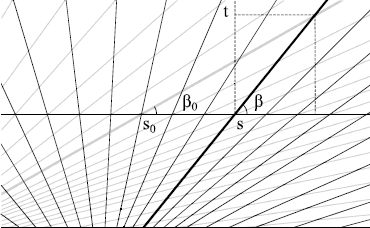Fig. 25

If η=η 0 in an isolated point then I 1 has a finite limit. To see this, let ηη 0=ϵ, |ϵ|≪1, at, say, s=0. Then we have s 0=t(ηη 0)= and lim ϵ→0 I 1(0,η)=2 0(0)(1+η 2(0))2, which means that the singularity is removable. If η=η 0 on an interval then s 0=s on that interval and the t-integration in Eq. (55) can be performed immediately, as in U 1.

### A.1 Two Special Cases

We consider two special cases where a reduction to a single integral over the centreline of the strip can be obtained explicitly.

• Cylindrical strip

If the relaxed shape of the strip is cylindrical, i.e., $$\eta_{0}=\operatorname{const}$$, then I 1 can be simplified to

$$I_1(s,\eta)=\frac{1}{\eta-\eta_0}\bigl[(1+ \eta_0\eta )^2 +\nu (\eta-\eta_0)^2\bigr] \int _{s_0^{-}}^{s_0^{+}} \kappa_0(s_0) \, \mathrm{d}s_0 ,$$

where $$s_{0}^{\pm}=s \pm w(\eta-\eta_{0})$$.

For a strip lying on a circular cylinder of constant radius R 0 and having a helical centreline with pitch angle $$\pi/2-\beta_{0}=\operatorname{const}$$., we have $$\kappa_{0} = \sin^{2}{\beta_{0}}/R_{0} =1/[R_{0} (1+\eta_{0}^{2})]=\operatorname{const}$$. and

$$U_2 = -\frac{2D}{R_0}\int_{0}^{L} \kappa \frac{(1+ \eta_0\eta )^2 +\nu (\eta-\eta_0)^2}{1+\eta_0^2}\, \mathrm{d}s$$

(cf. ).

• Corrugated strip

Now let κ 0 be a prescribed periodic function, for instance κ 0(s)=Asin(υs), where the amplitude A and frequency υ are constant. Taking for simplicity η 0≡0, then

$$U_2=- \frac{2DA}{\upsilon} \int_{0}^{L} \frac{\kappa \sin(\upsilon w\eta)}{\eta} \bigl(1+\nu\eta^2\bigr) \sin(\upsilon s)\, \mathrm{d}s .$$

We note that the integrand now explicitly depends on arclength s.

### Appendix B: The Conical Limit

Here we explore the particular (singular) case in which the developable strip adopts a conical shape. The statics of conical surfaces has attracted extensive attention recently [5, 6, 18], especially in models for paper crumpling. Approximately conical pieces appear in our computed solutions for relatively wide strips. Our particular aim here is to describe the limiting tri-conical shape of the D 3-symmetric one-sided strip with Lk=3/2 as the strip’s aspect ratio is increased (see Fig. 19).

The limiting strip solution that we are interested in is assembled from six congruent conical pieces. It is sufficient to focus on finding the shape of one such piece for $$s \in [0, w\sqrt{2}]$$. The edge of regression for this conical piece degenerates into a point, the apex of the cone. The condition for all generators to go through this point, $$\eta=\sqrt{2}-s/w$$, gives an explicit formula for η (see Fig. 26). This η makes the logarithm in the bending energy density, Eq. (9), divergent. We regularise this energy by cutting off a small triangular domain near the apex of the cone. Thus we compute the bending energy by performing integration in Eq. (7) for t∈[−w,(1−ϵ)w], 0<ϵ≪1 (we can justify this by appealing to the fact that the local curvature becomes too high near the apex for the assumption of an elastic material to remain valid ). Then V is a multiplicative constant, $$V=-\frac{1}{2} \log{\frac{\epsilon}{2}}$$, in Eq. (9) and therefore does not enter the equilibrium equations. To account for the conical η(s) given above we replace the density h(κ,η,η′) in Eq. (8) with

$$\tilde{h}(\kappa,\eta)=\kappa^2 \bigl(1+\eta^2 \bigr)^2 + \lambda(s) (\eta-\sqrt{2}+s/w ),$$

where λ is a Lagrange multiplier. Note that this is exactly Sadowsky’s density but with prescribed η.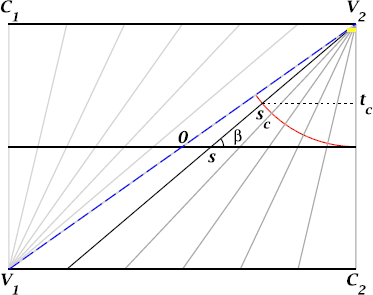Fig. 26

The equations for this density follow from the procedure described in Sects. 4.1 and 4.2. The constitutive Eq. (21) allows us to express

$$\kappa = \frac{\eta M_{1}+M_{3}}{2(1+\eta^2)^2},$$
(56)

which we substitute into the balance equations to obtain a closed system of six non-autonomous equations, Eqs. (16), (17), for the six components (F 1,F 2,F 3,M 1,M 2,M 3). We can then formulate a boundary-value problem as in Sect. 6.1 on the interval $$[0,w\sqrt{2}]$$ to find the forces, moments and the curvature of the centreline and reconstruct the surface of the strip. There is however one difference and that is that for the η as prescribed we cannot satisfy the boundary condition η′(0)=0. This means that the resulting assembled strip has only a continuous (and generally not a differentiable) generator at s=0. All other boundary conditions carry over to the present limiting situation. In particular, the condition $$\kappa(w\sqrt{2})=0$$ follows from Eq. (56), the fact that $$\eta(w\sqrt{2})=0$$ and the other condition $$M_{3}(w\sqrt{2})=0$$. The remaining constitutive Eq. (22) may be used to obtain the Lagrange multiplier λ(s). However, instead of pursuing this formulation it is instructive for this conical problem to use a different formulation as we discuss next.

The bending energy of the cone has a particularly simple form if we change to a different reference curve, namely the circular arc of radius w centred at the apex of the cone (see Fig. 26). Let s c be the arclength coordinate along this arc. It is easy to see that the generators induce a one-to-one mapping between the strip’s centreline and the circular arc, ss c for $$s \in [0, w\sqrt{2}]$$, s c ∈[0,s ce ], $$s_{ce}=w\arctan{\sqrt{2}}$$, given by $$\sqrt{2}-\frac{s}{w}=\tan{\frac{s_{ce}-s_{c}}{w}}= \tan(\arctan(\sqrt{2})-\frac{s_{c}}{w})$$ and ds=(1+η 2) ds c . Let κ N be the normal curvature of the new reference curve. It equals the nonzero principal curvature at the same point, which we can compute from Eq. (2) by noting that t is now given by the value t c at the intersection of the local generator with the circular arc (see Fig. 26). We have $$t_{c}=w(1-\sin\beta)=w(1-1/\sqrt{1+\eta^{2}})$$ and hence κ N =−κ(1+η 2)3/2. We can then express the bending energy as

$$\int_0^{w\sqrt{2}} \kappa(s)^2 \bigl(1+ \eta(s)^2 \bigr)^2 \,\mathrm{d} s = \int _0^{s_{ce}} \kappa_N^2 (s_c) \,\mathrm{d} s_c .$$

This simple form suggests that the equation governing the normal curvature is that of the Euler elastica, as observed previously in . To demonstrate this we introduce the Darboux frame {T,N,U} at the reference arc with the unit tangent T, normal to the surface N and U=T×N. Then we define R c (s c ):=(T,N,U)∈SO(3) and $$\mathsf{\widehat{\omega}}_{c}=R_{c}^{\intercal}\frac{\mathrm{d} R_{c}}{\mathrm{d} s_{c}} \in \mathfrak{so}(3)$$, so that ω c1=τ g , ω c2=κ g , ω c3=κ N (we omit the subindex ‘c’ in τ g , κ g and κ N ). Since our circular arc lies on the conical surface with tangent orthogonal to the generator everywhere, we have τ g ≡0 and κ g ≡1/w. Then we define the reduced Lagrangian as

$$\mathcal{L}_c = \omega_{c3}^2 + M_{c1}\omega_{c1} + M_{c2} \biggl( \omega_{c2} - \frac{1}{w} \biggr) - F_{c1} ,$$

where M c1(s c ) and M c2(s c ) are Lagrange multipliers. Applying again the procedure described in Sect. 4.1, we obtain the six balance equations for (F c1,F c2,F c3,M c1,M c2,M c3) and the constitutive equation

$$M_{c3} = 2\kappa_N.$$
(57)

For completeness we give the relationship between the system of forces and moments at the centreline and the equivalent transferred system of forces and moments at the circular arc:

\begin{aligned} F_{c1} =& \frac{1}{\sqrt{1+\eta^2}} (F_1 - \eta F_3 ), \\ F_{c2} =& - F_2, \\ F_{c3} =& - \frac{1}{\sqrt{1+\eta^2}} (\eta F_1 + F_3 ), \\ \\ M_{c1} =& \frac{1}{\sqrt{1+\eta^2}} (M_1 - \eta M_3 ) + w \bigl(\sqrt{1+\eta^2} -1 \bigr) F_{2}, \\ M_{c2} =& - M_2 + w \biggl(1-\frac{1}{\sqrt{1+\eta^2}} \biggr) (F_1-\eta F_3 ), \\ M_{c3} =& - \frac{1}{\sqrt{1+\eta^2}} (\eta M_1 + M_3 ) . \end{aligned}
(58)

We can also write the Hamiltonian corresponding to the Lagrangian $$\mathcal{L}_{c}$$:

$$\mathcal{H}_c=\kappa^2_N + \frac{M_{c2}}{w}+F_{c1},$$
(59)

which is a conserved quantity. (By using Eqs. (58) this Hamiltonian when expressed in terms of centreline variables becomes $$\mathcal{H}_{c} =\kappa^{2} (1+\eta^{2})^{3} - \frac{M_{2}}{w}+F_{1}-\eta F_{3}$$. That this is a conserved quantity, despite the fact that η is a given function of s, can also be verified by differentiation and using the balance equations for the centreline forces and moments.)

From the first and last moment balance equations we find $$M_{c2}=\frac{1}{\kappa_{N}} \frac{\mathrm{d} M_{c1}}{\mathrm{d} s_{c}} + \frac{2}{w}$$ and $$F_{c2}= \frac{M_{c1}}{w} - 2 \frac{\mathrm{d} \kappa_{N}}{\mathrm{d} s_{c}}$$. Differentiating the latter and substituting the result together with F c1 obtained from Eq. (59) into the second force balance equation we arrive at

$$\frac{\mathrm{d}^2 \kappa_N}{\mathrm{d} s_c^2}+\frac{\kappa_N}{2} \biggl(\kappa_N^2 - H_c + \frac{2}{w^2} \biggr) = 0 ,$$
(60)

where H c is the value of the Hamiltonian $$\mathcal{H}_{c}$$, as yet undetermined. This is the curvature equation for the planar Euler elastica, which has well-known solutions in terms of Jacobi elliptic functions.

To reconstruct the surface, we first parametrise the vectors with three Euler angles ψ c , ϑ c , φ c :

\begin{aligned} \boldsymbol{T} =& \begin{pmatrix} -\sin\psi_c \sin\varphi_c+\cos\vartheta_c \cos\psi_c \cos\varphi_c \\ \cos\psi_c \sin\varphi_c +\cos\vartheta_c \sin\psi_c \cos\varphi_c \\ -\sin\vartheta_c \cos\varphi_c \end{pmatrix}, \\ \boldsymbol{N} =& \begin{pmatrix} -\sin\psi_c \cos\varphi_c-\cos\vartheta_c \cos\psi_c \sin\varphi_c \\ \cos\psi_c \cos\varphi_c -\cos\vartheta_c \sin\psi_c \sin\varphi_c \\ \sin\vartheta_c \sin\varphi_c \end{pmatrix} , \qquad \boldsymbol{U}= \begin{pmatrix} \sin\vartheta_c \cos\psi_c \\ \sin\vartheta_c \sin\psi_c \\ \cos\vartheta_c \end{pmatrix} . \end{aligned}

The Euler angles are related to the Darboux vector by the kinematics equations

\begin{aligned} \frac{\mathrm{d} \psi_c}{\mathrm{d} s_c} =& - \frac{1}{w}\sin\varphi_c \csc \vartheta_c , \\ \frac{\mathrm{d} \vartheta_c}{\mathrm{d} s_c} =& -\frac{1}{w}\cos\varphi_c , \\ \frac{\mathrm{d} \varphi_c}{\mathrm{d} s_c} =& \kappa_N +\frac{1}{w}\sin \varphi_c \cot\vartheta_c . \end{aligned}
(61)

Note that the angle ϑ c should not approach 0 or π. Finally, to find the reference arc r c =(x,y,z) in three-dimensional space we solve Eq. (61) in conjunction with the equation $$\frac{\mathrm{d} \boldsymbol{r}_{c}}{\mathrm{d} s_{c}} = \boldsymbol{T}$$.

To compute limiting tri-conical shapes of our one-sided strip we solve the elastica equation (60) together with Eq. (61) on the interval [0,s ce ] subject to appropriate boundary conditions. We choose an orientation such that the tangent T(0) is aligned with the z-axis and the vectors U(0) and U(s ce ) span the xy-plane, making an angle of π/6 (cf. Eq. (52)). The xy-plane will then be a symmetry plane for the full strip. We also require the normal curvature to vanish at s ce . All this translates into the boundary conditions

\begin{aligned} &\phantom{\qquad\qquad\ \ \ \,} \kappa_N(s_{ce}) =0 , \\ \psi_c(0) =&-\pi/6,\qquad \psi_c(s_{ce}) =0, \\ \vartheta_c(0) =& \pi/2,\qquad\quad \vartheta_c(s_{ce}) =\pi/2, \\ \varphi_c(0) =& 0 , \end{aligned}

i.e., six boundary conditions for a fifth-order system of equations and one free parameter H c . Since the parameter w can be trivially scaled out the solution to this boundary-value problem yields a universal tri-conical shape for our strip. Numerical solution gives H c w 2=−1.830652 and the graphs for the normal curvature κ N on the circular arc and the curvature κ of the centreline shown in Fig. 27. Figure 28 illustrates the assembly of the entire shape from a single piece in the same manner as described in Sect. 6.3. The resulting shape is barely distinguishable from the shape in Fig. 19 (Fig. 20 compares η for the two solutions). Note that while the normal to the surface is continuous everywhere, the curvature is discontinuous at the generators connecting the apices.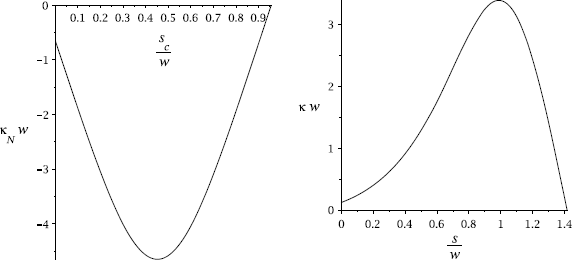Fig. 27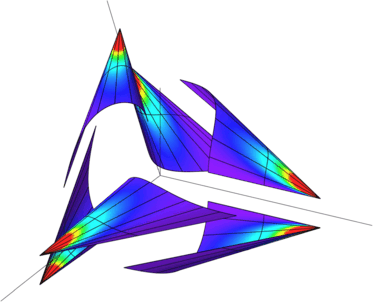Fig. 28

The shape of the conical strip has been found without simultaneously solving for the forces and moments whose equations are semi-decoupled from the elastica equation (60). Once κ N has been found the force and moment equations form a system of six linear equations for the components F c1, F c2, F c3, M c1, M c2 and M c3. We can solve these equations subject to our standard force and moment conditions, Eqs. (40) and (41), translated into conditions for the above components by using the relationships (58). These four conditions, together with the single constitutive equation (57) and the Hamiltonian (59), both of which are fixed by the geometry, yield a unique solution.

## Rights and permissions

Open Access This article is distributed under the terms of the Creative Commons Attribution License which permits any use, distribution, and reproduction in any medium, provided the original author(s) and the source are credited.

Reprints and Permissions